出租广告位,需要合作请联系站长

+关注

2020-07(11)

2020-08(42)

# 一、模型层

## 1.1. 模型层定义

Django MTV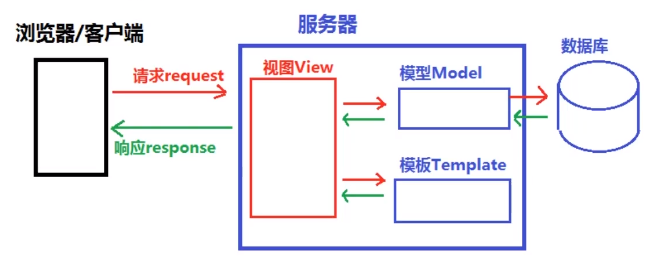## 1.2. Django配置MySQL

• 安装`mysqlclient`【版本mysqlclient 1.3.13以上，官网目前为2.0.x】

• 安装前确认Ubuntu是否已安装`python3-dev``default-libmysqlclient-dev`

1. `sudo apt list --installed|grep -E 'libmysqlclient-dev|python3-dev'`
2. 若命令无输出则需要安装 - `sudo apt-get install python3-dev default-libmysqlclient-dev`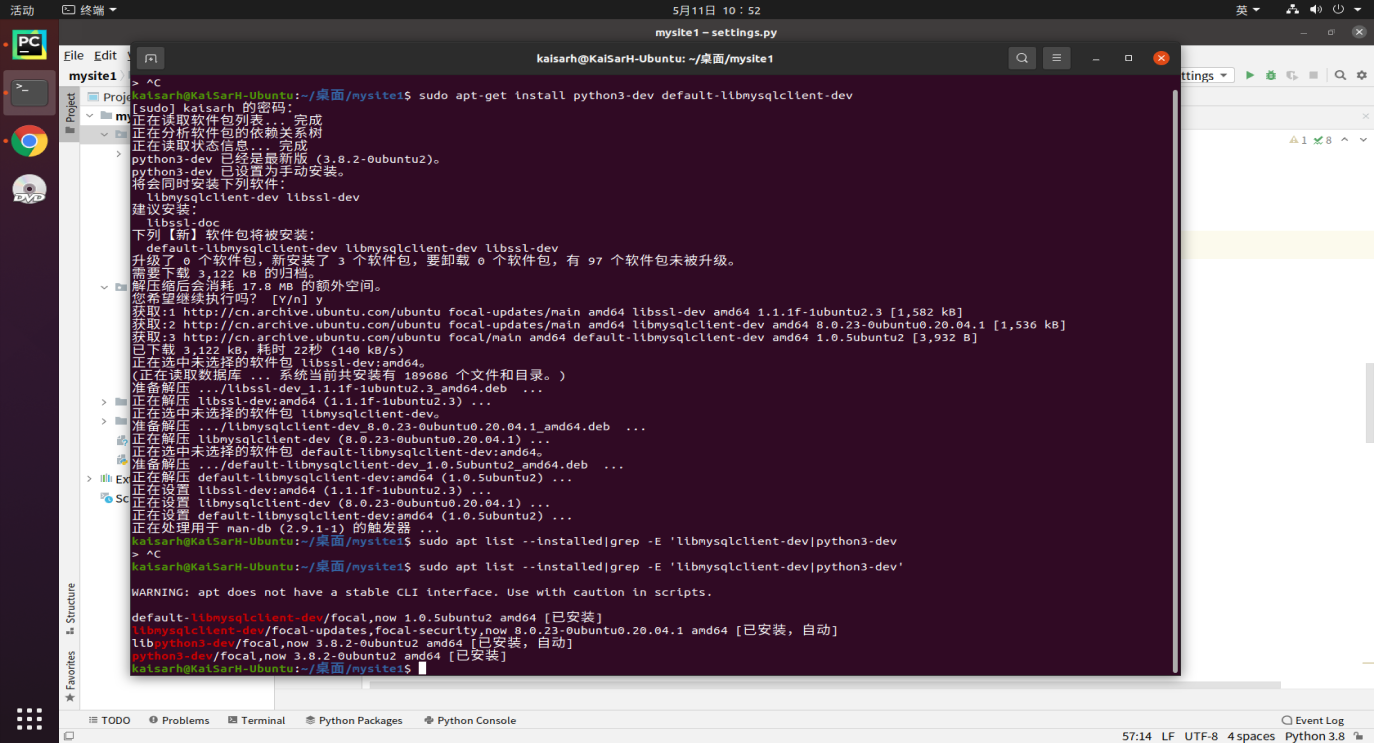• 安装`mysqlclient``sudo pip3 install mysqlclient`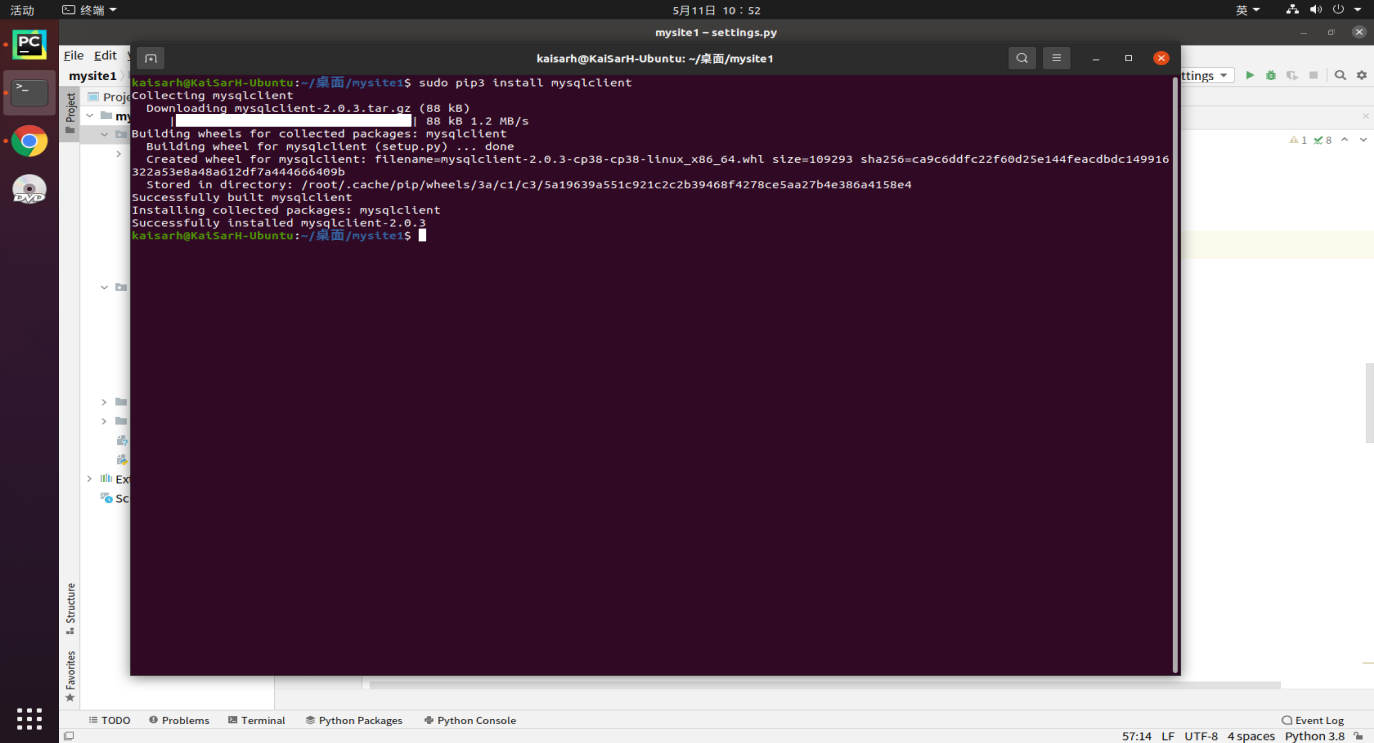• 检查`sudo pip3 freeze|grep -i 'mysql'`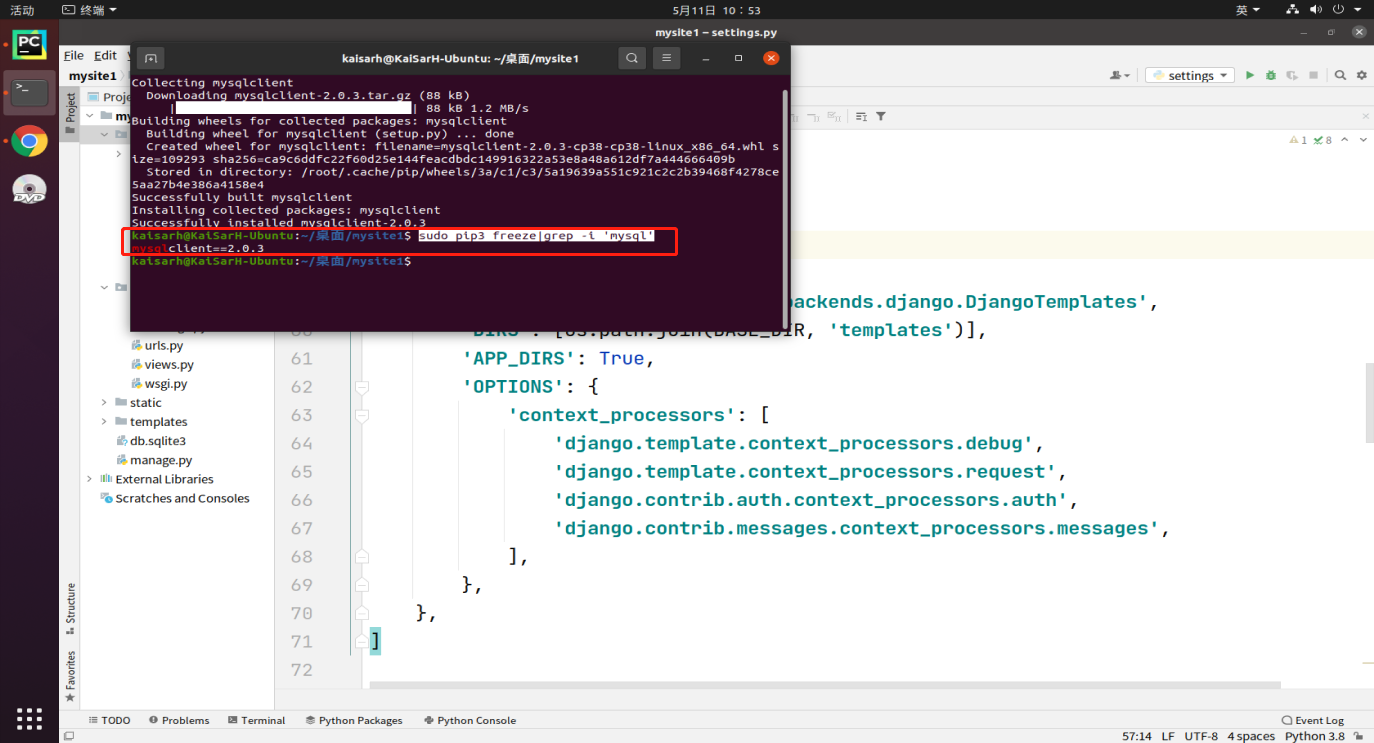## 1.3. 创建数据库

``````create database 数据库名 default charset utf8
``````

## 1.4. 配置数据库

setting.py里进行数据库的配置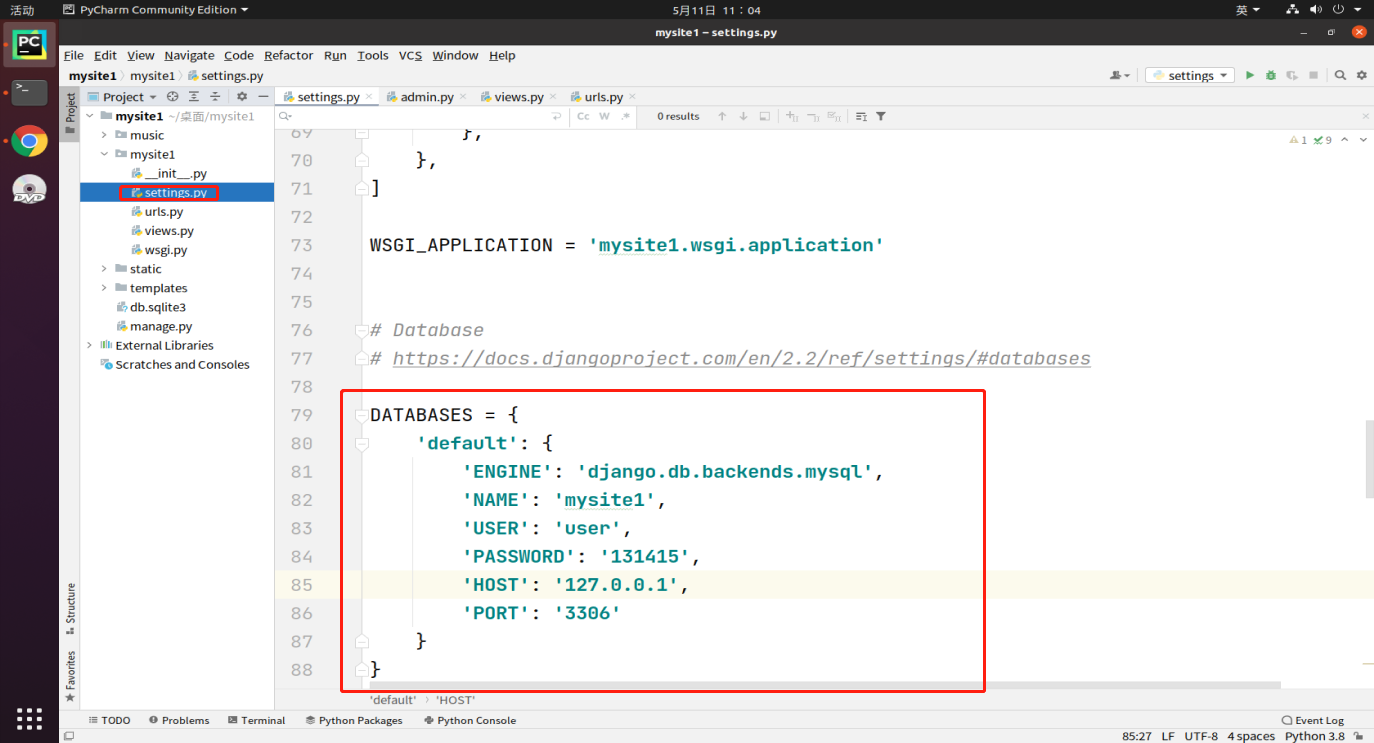• ENGINE：指定数据库存储引擎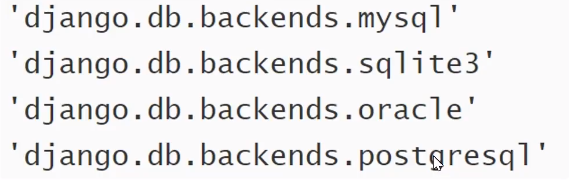• NAME：指定要连接的数据库的名称

• USER：指定登录到数据库的用户名

• HOST/PORT：连接具体数据库的IP和端口

## 1.5. 模型

• 模型是一个Python类，它是由django.db.models.Model派生出的子类
• 一个模型类代表数据库中的一张数据表
• 模型类中每一个类属性都代表数据库中的一个字段
• 模型是数据交互的接口，是表示和操作数据库的方法和方式

# 二、ORM框架

## 2.1. 定义

ORM（Object Relational Mapping）即对象关系映射，它是一种程序技术，它允许你使用类和对象对数据库进行操作，从而避免通过SQL语句操作数据库

## 2.2. 作用

1. 建立模型类和表之间的对应关系，允许我们通过面向对象的方式来操作数据库
2. 根据设计的模型类生成数据库中的表格
3. 通过简单的配置就可以进行数据库的切换

## 2.3. 优点

1. 只需要面向对象编程，不需要面向数据库编写代码。

• 对数据库的操作都转换成对类属性和方法的操作
• 不用编写各种数据库的sql语句
2. 实现了数据模型与数据库的解耦，屏蔽了不同数据库操作上的差异。

• 不再关注用的是MySQL、Oracle…等数据库的内部细节
• 通过简单的配置就可以轻松更换数据库，而不需要修改代码

## 2.4. 缺点

1. 对于复杂业务，使用成本较高
2. 根据对象的操作转换成SQL语句，根据查询的结果转换成对象，在映射过程中有性能损失

## 2.5. 映射图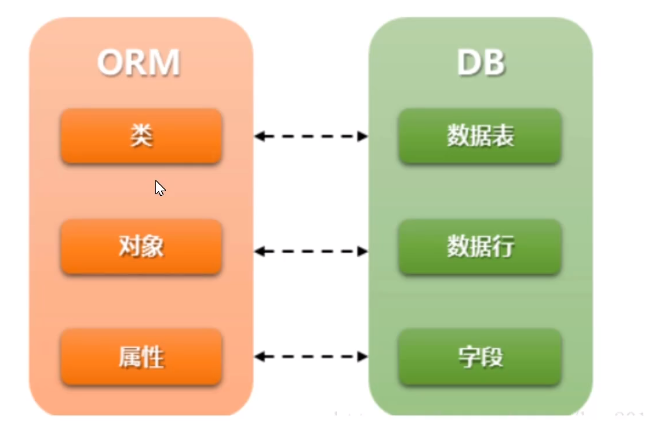## 2.6. 模型示例

1. 添加一个bookstore的app

`python3 manage.py startapp bookstore`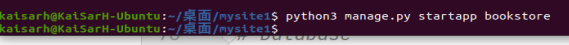2. 注册应用app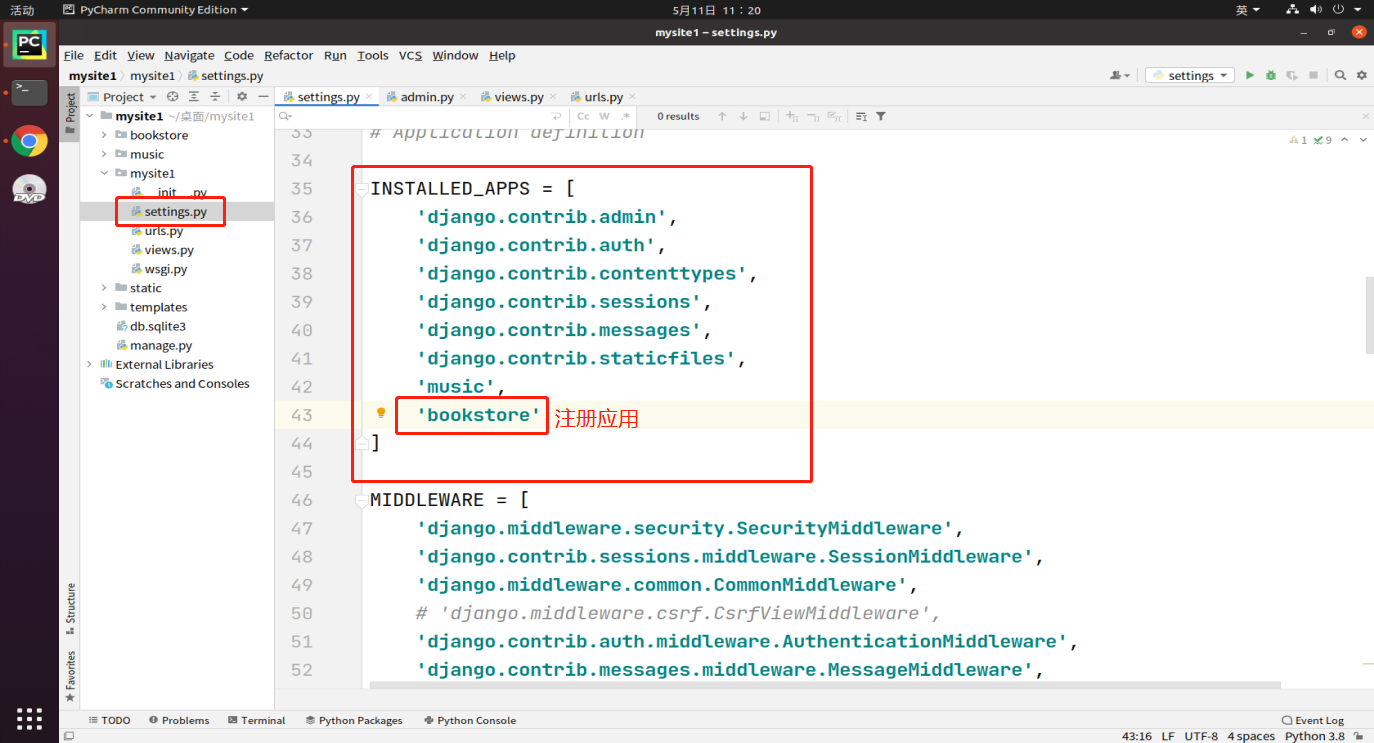3. 添加模型类

模型类代码示例

``````# file : bookstore/models.py
from django.db import models

class Book(models.Model):
title = models.CharField("书名", max_length=50, default='')
price = models.DecimalField("定价", max_digits=7, decimal_places=2, default=0.0)
``````
4. 数据库迁移

迁移是Django同步对模型所做的更改（添加字段、删除模型等）到数据库模式的方式

• 生成迁移文件 — 执行 python3 manage.py makemigrations

将应用下的models.py文件生成一个中间文件，并保存在migrations文件夹中

• 执行迁移脚本程序 — 执行 python3 manage.py migrate

执行迁移程序实现迁移。将每个应用下的migrations目录中的中间文件同步回数据库

5. 效果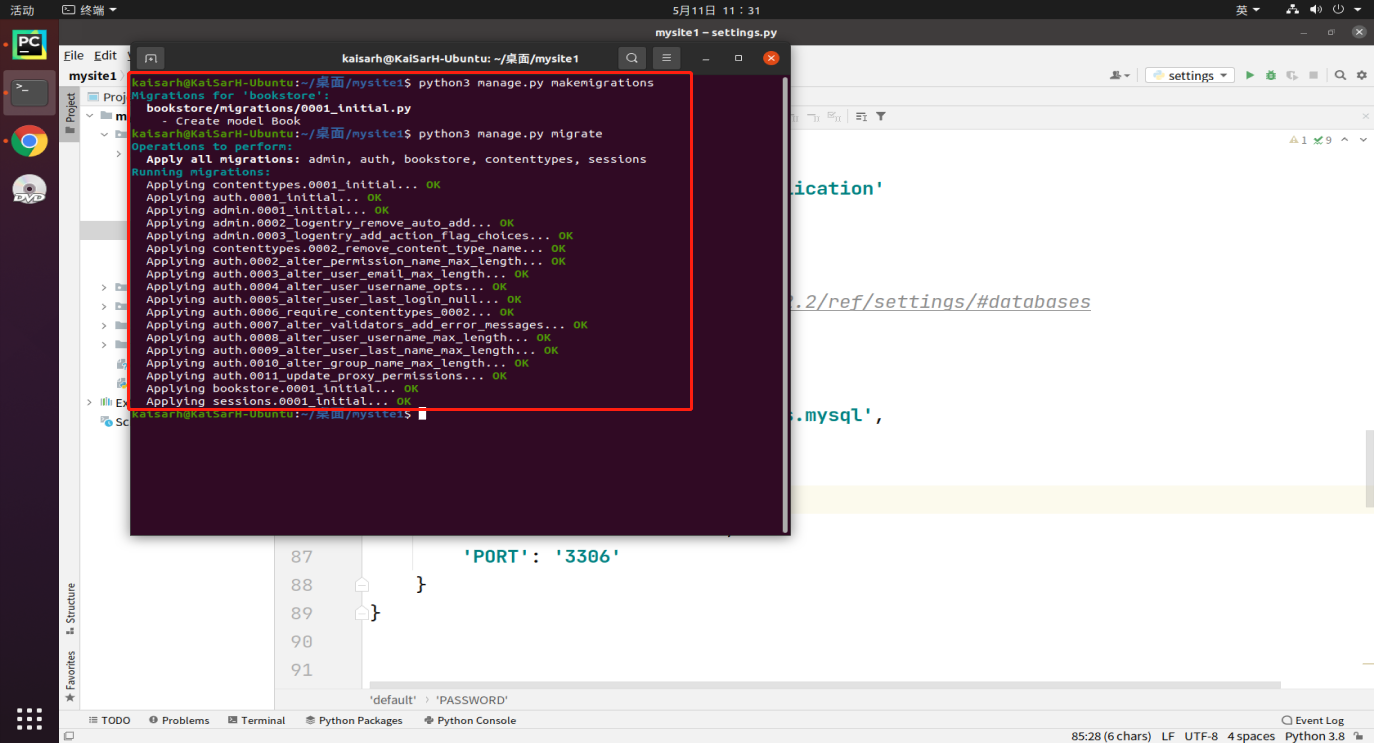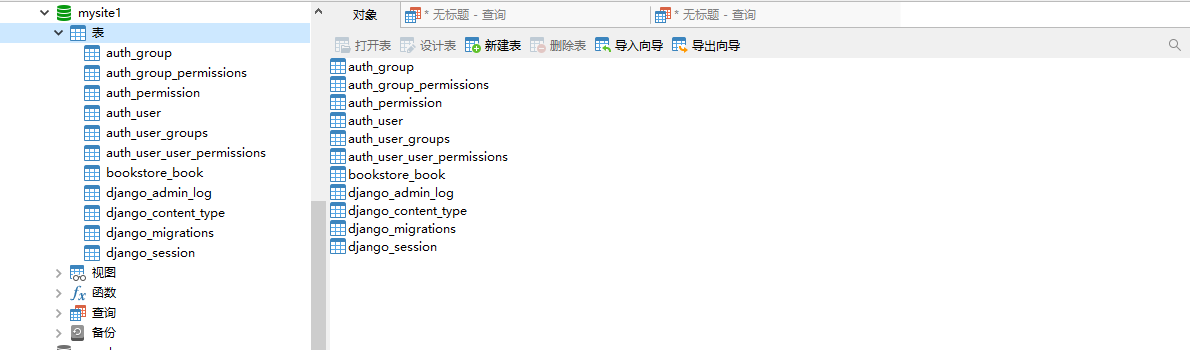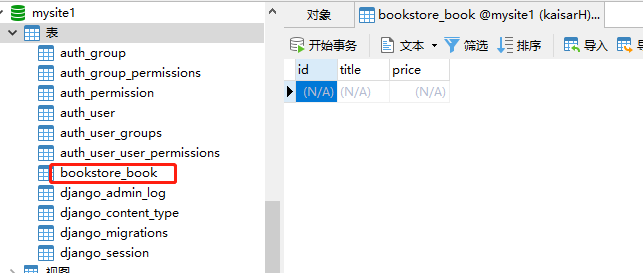# 三、模型类

## 3.1. 创建

• 创建应用

• 在应用下的models.py中编写模型类

``````from django.db import models
class 模型类名(models.Model):
字段名 = models.字段类型(字段选项)
``````
• 迁移同步 makemigrations & migrate

• 任何关于表结构，务必在对应模型类上修改

例：为bookstore_book表添加一个名为info的字段varchar(100)

解决方案：

• 模型类中添加对应类属性
• 执行数据库迁移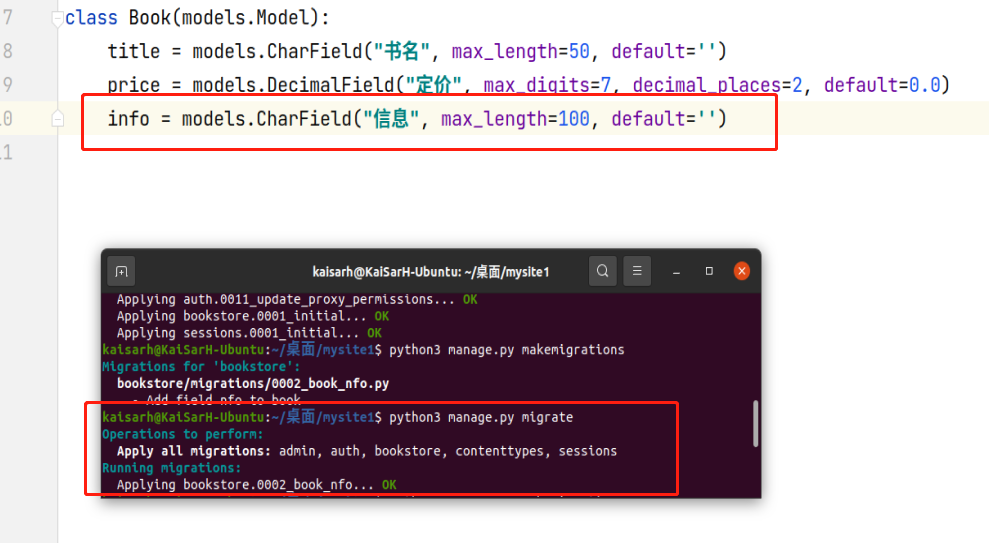## 3.2. 基础字段

• **BooleanField() **

• 数据库类型：tinyint(1)
• Django类型：使用True或False来表示值
• 在数据库中，使用1或者0来表示具体的值
• CharField()

• 数据库类型：varchar
• 注意： 必须要指定max_length参数值
• DateField()

• 数据库类型：date
• 作用：表示日期
• 参数：
• auto_now：每次保存对象时，自动设置该字段为当前时间（取值：True / False）
• default：设置当前时间（取值：字符串格式时间，如：‘2019-6-1’）
• 以上三个参数只能多选一
• DateTimeField()

• 数据库类型：datetime(6)
• 作用：表示日期和时间
• 参数同DateField
• FloatField()

• 数据库类型：double
• Django、数据库中都是用小数表示值
• DecimalField()

• 数据库类型：decimal(x,y)

• Django、数据库中都是用小数表示值

• 参数：

• max_digits：位数总数，包括小数点后的位数。该数值必须大于等于decimal_places
• decimal_places：小数点后的数字量数
• EmailField()

• 数据库类型：varchar
• Django、数据库中都是用字符串表示值
• IntegerField()

• 数据库类型：int
• Django、数据库中都是用整数表示值
• ImageField()

• 数据库类型：varchar(100)
• 作用：在数据库中为了保存图片的路径
• Django、数据库中都是用字符串表示值
• TextField()

• 数据库类型：longtext
• 作用：表示不定长的字符数据
• 官方文档

https://docs.djangoproject.com/en/2.2/ref/models/fields/#field-types

## 3.3. 创建模型类测试

• Author - 作者

• name CharField 姓名 长度最大11
• age IntegerField 年龄
• email EmailField 邮箱
• 代码：

``````class Author(models.Model):
name = models.CharField("姓名", max_length=11)
age = models.IntegerField("年龄")
email = models.EmailField("邮箱")
``````
• 迁移：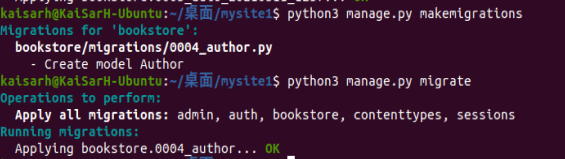## 3.4. 字段选项

• 字段选项，指定创建的列的额外的信息

• 允许出现多个字段选项，多个选项之间使用`,`隔开

• primary_key：如果设置为True，表示该列为主键，如果指定一个字段为逐渐，则此数据表不会创建id字段。

• blank：设置为True时，字段可以为空。设置为False时，字段是必须填写的。

• null：如果设置True，表示该列值允许为空。默认为False，如果此选项为False，建议加入default选项来设置默认值。

• default：设置所在列的默认值，如果字段选项null=False，建议添加此项。

• db_index：如果设置为True，表示为该列增加索引。

• unique：如果设置为True，表示该字段在数据库中的值必须是唯一（不能重复出现）。

• db_column：指定列的名称，如果不指定的话则采用属性名作为列名。

## 3.5. 字段选项样例

``````# 创建一个属性，表示用户长度，长度30个字符，必须是唯一的，不能为空，添加索引
name = models.CharField(max_length=30, unique=True, null=False, db_index=True)
``````

https://docs.djangoproject.com/en/2.2/ref/models/fields/#field-options

## 3.7. Meta类

``````# file : bookstore/models.py
from django.db import modele
class Book(models.Model):
title = models.CharField("书名", max_length=50, default='')
price = models.DecimalField("定价", max_digits=7, decimal_places=2, default=0.0)
info = models.CharField("信息", max_length=100, default='')

class Meta:
db_table = 'book'  # 可改变当前模型类对应的表名
``````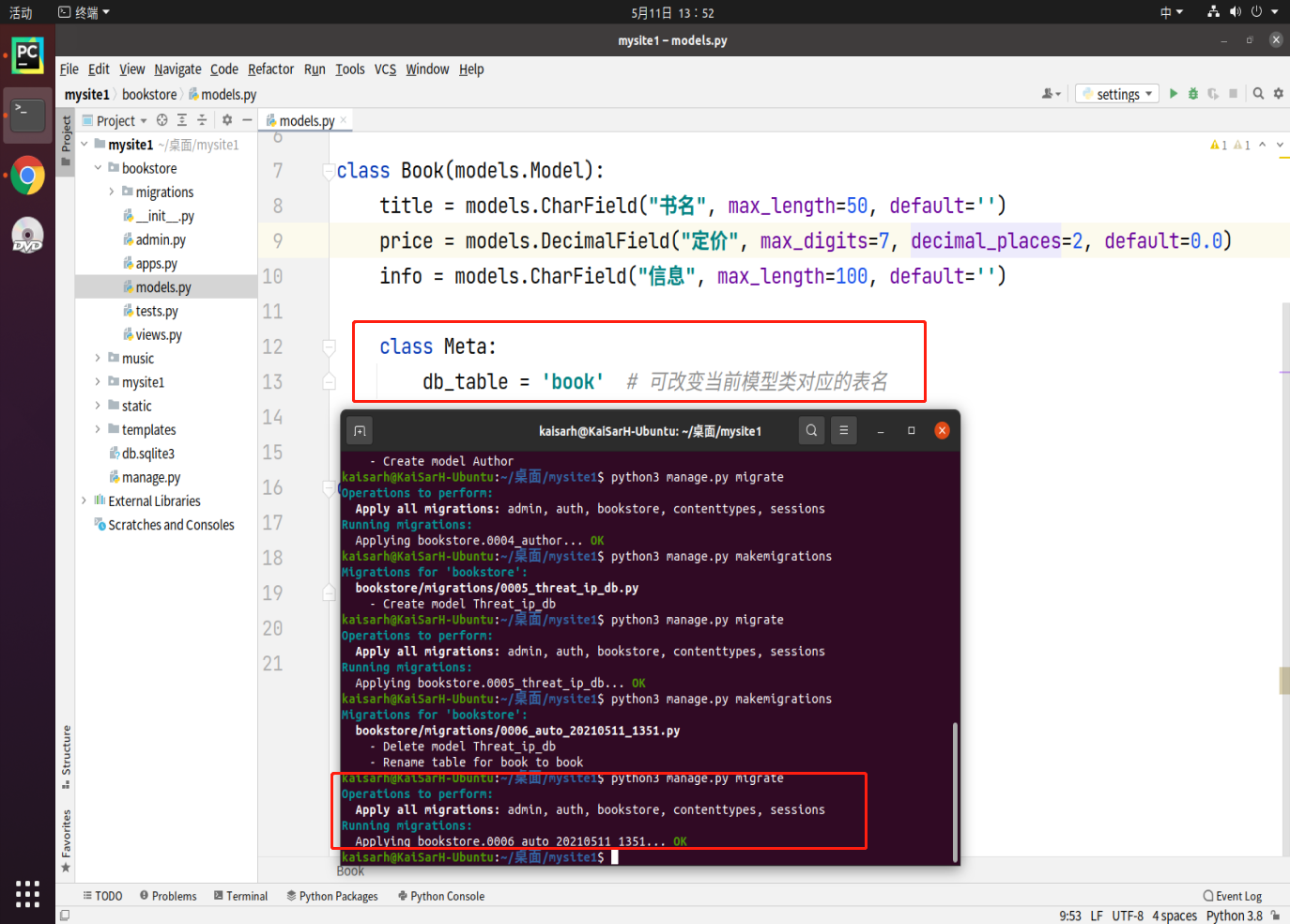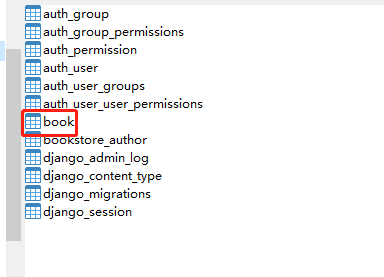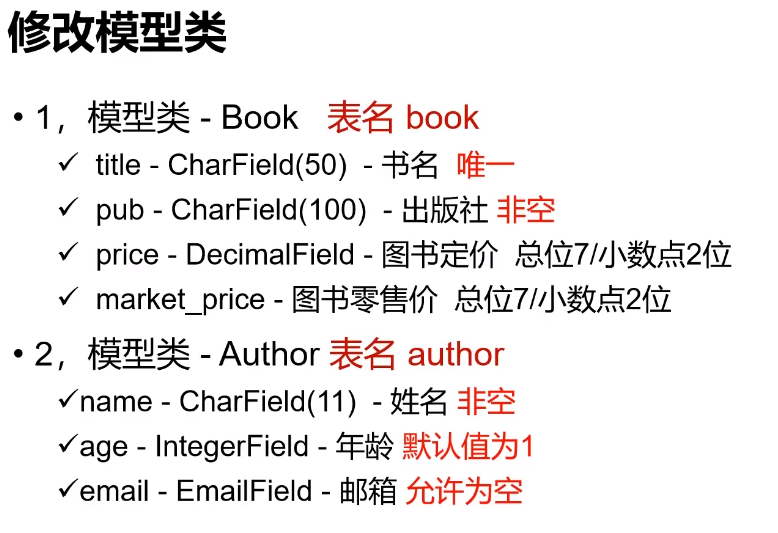``````class Book(models.Model):
title = models.CharField("书名", max_length=50, default='', unique=True)
pub = models.CharField("出版社", max_length=100, default='', null=False)
price = models.DecimalField("价格", max_digits=7, decimal_places=2)
marker_price = models.DecimalField("零售价", max_digits=7, decimal_places=2, default=0.0)

class Meta:
db_table = 'book'  # 可改变当前模型类对应的表名

class Author(models.Model):
name = models.CharField("姓名", max_length=11, null=False)
age = models.IntegerField("年龄", default="1")
email = models.EmailField("邮箱", null=True)

class Meta:
db_table = 'author'  # 可改变当前模型类对应的表名
``````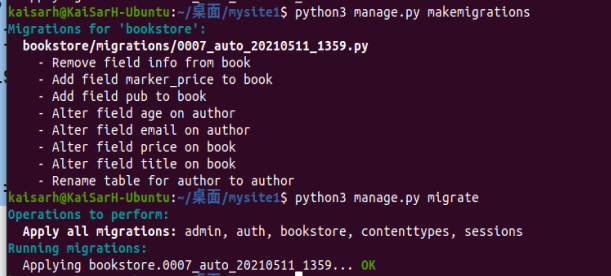# 四、常见问题及解决方法

1. 问题1：当执行`python3 manage.py makemigrations`出现如下迁移错误时的处理方法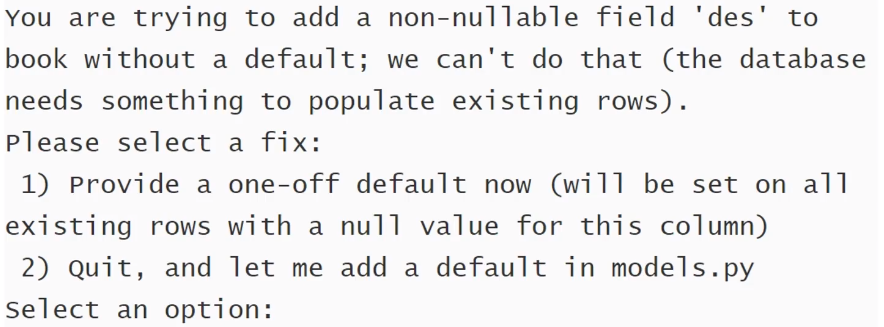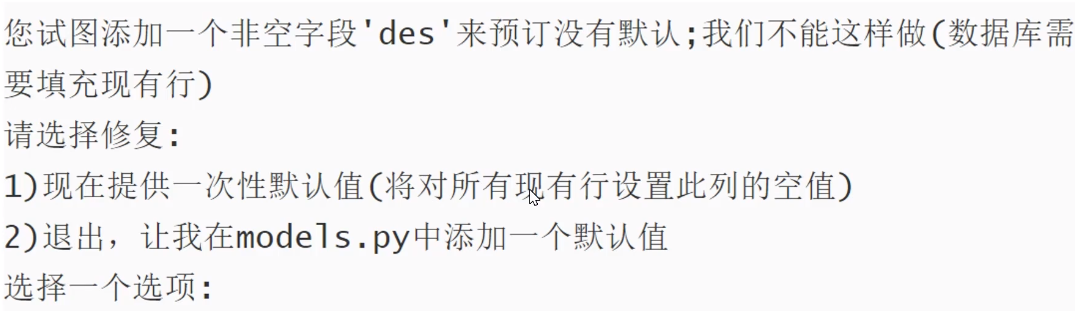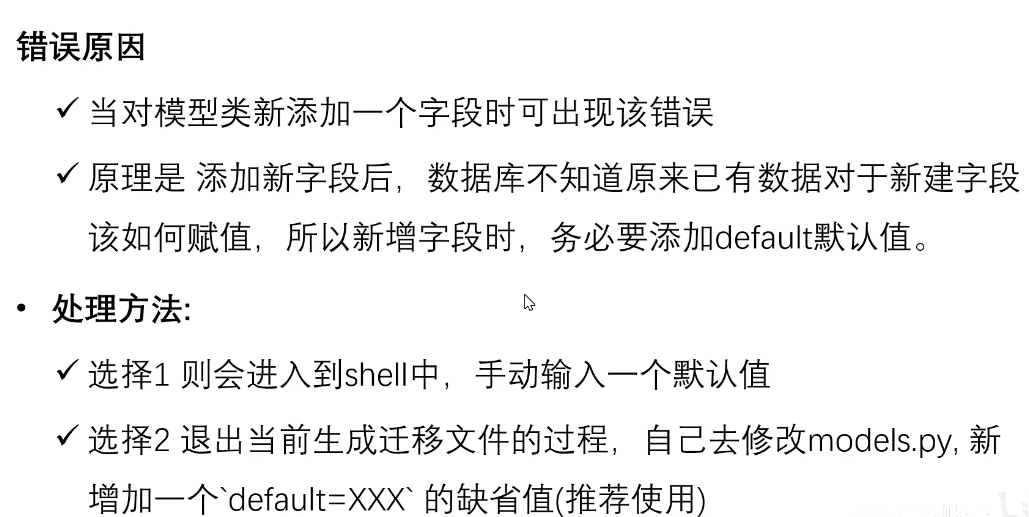2. 数据库的迁移文件混乱的解决方法

• 数据库中django_migrations表记录了migrate的’全过程’，项目个应用中的migrate文件与之对应，否则migrate会报错。

• 解决方案：

1. 删除所有migrations里所有的000?XXXX.py（__init_.py除外）

2. 删除数据库

``````drop database mywebdb;
``````
3. 重新创建数据库

``````create database mywebdb default charset...;
``````
4. 重新生成migrations里面所有的000?_XXXX.py

``````python3 manage.py makemigrations
``````
5. 重新更新数据库

``````python3 manage.py migrate
``````

# 五、Django Shell

Django提供了一个交互式的操作项目叫Django Shell，它能够在交互模式用项目工程的代码执行相应的操作

``````python3 manage.py shell
``````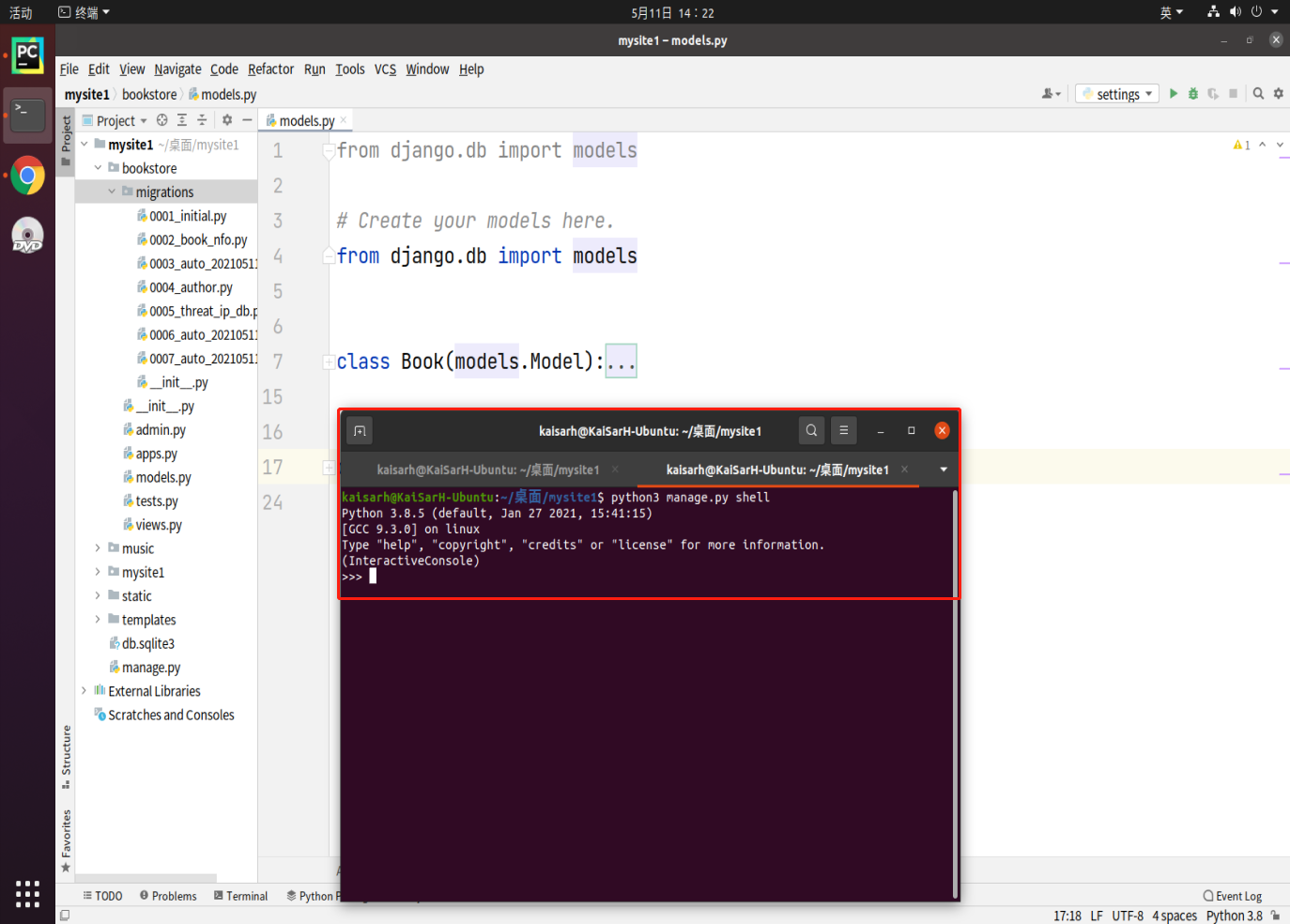# 六、ORM操作

ORM CURD核心 → 模型类.管理器对象

## 6.1. 管理器对象

``````class MyModel(models.Model):
···
MyModel.objects.create(...) # objects 是管理器对象
``````

## 6.2. 创建/插入数据

Django ORM使用一种直观的方式把数据库表中的数据表示成Python对象，创建数据中每一条记录就是创建一个数据对象

### 6.2.1. 方案1

``````MyModel.objects.create(属性1=值1,属性2=值2...)
``````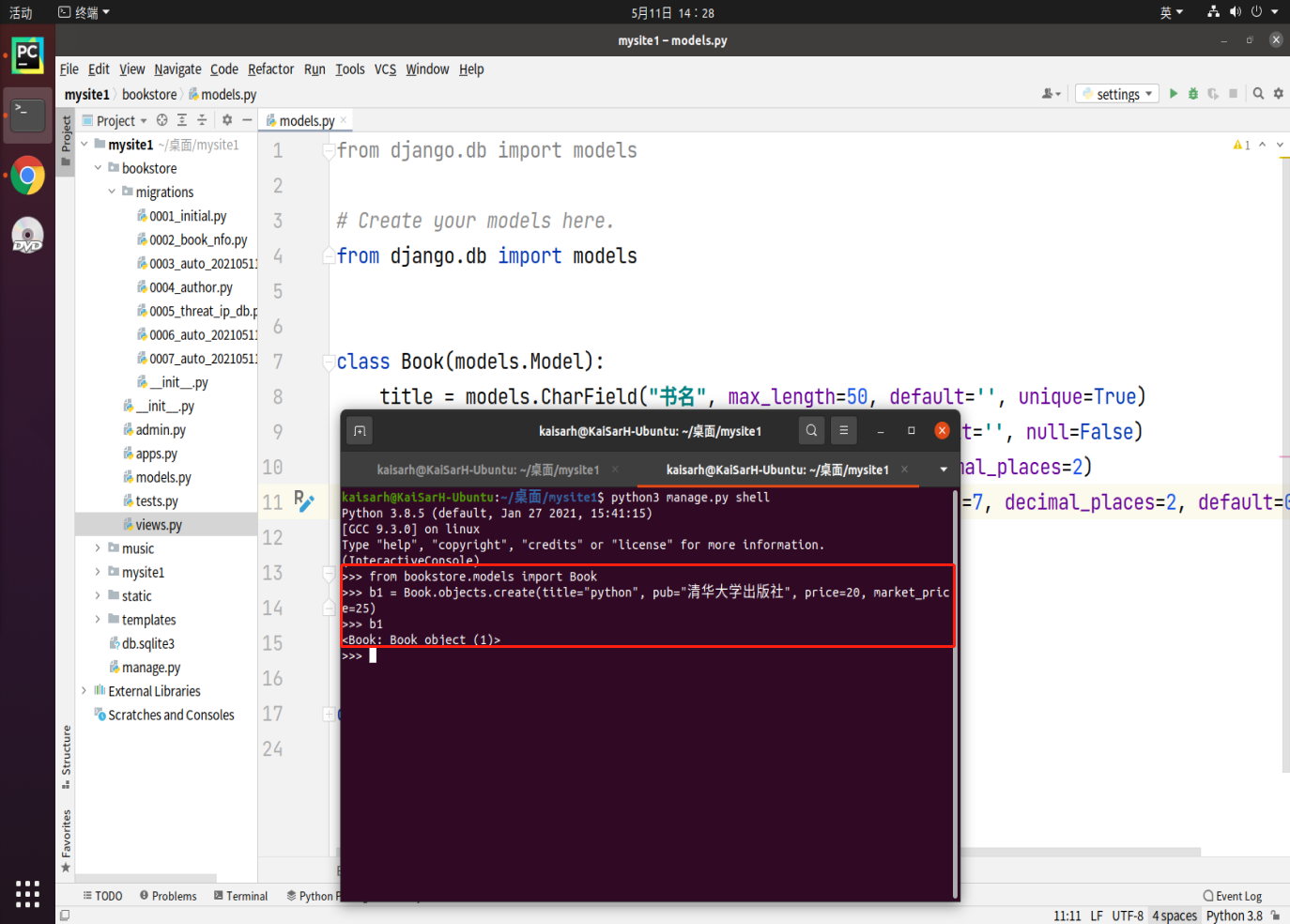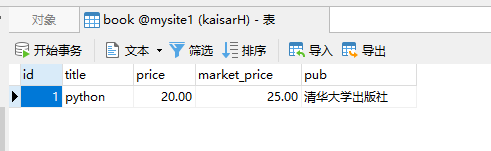### 6.2.2. 方案2

``````obj = MyModel(属性=值,属性=值..)
obj.属性 = 值
obj.save()
``````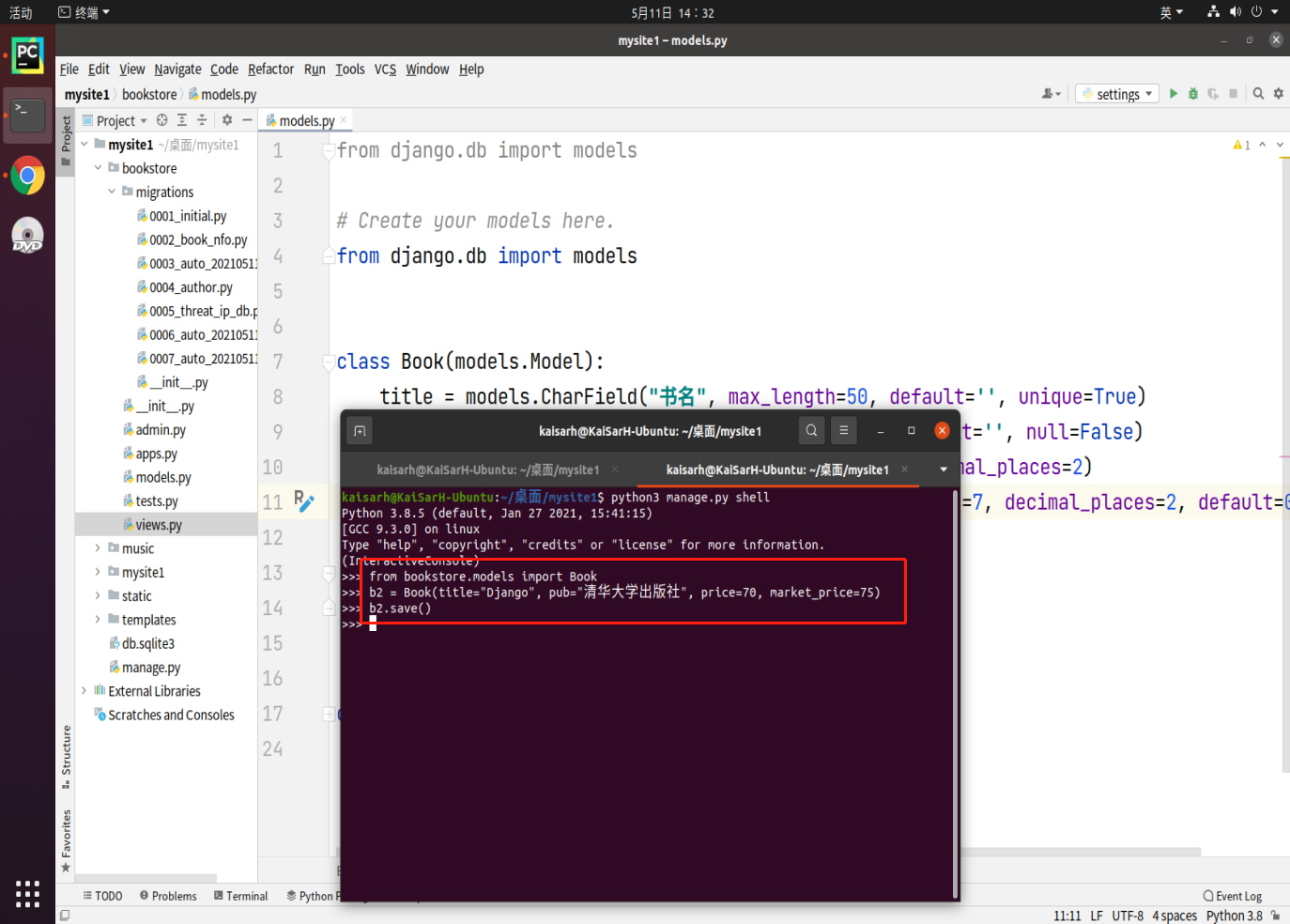### 6.2.3. 练习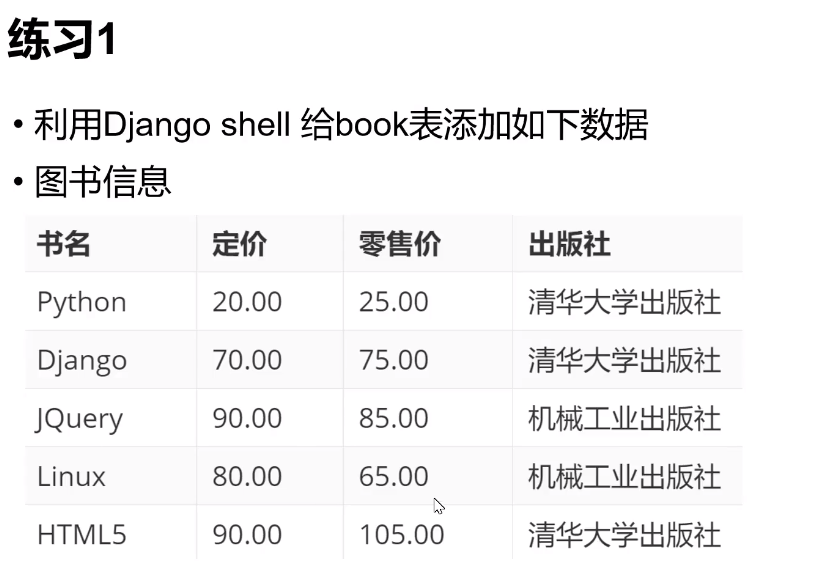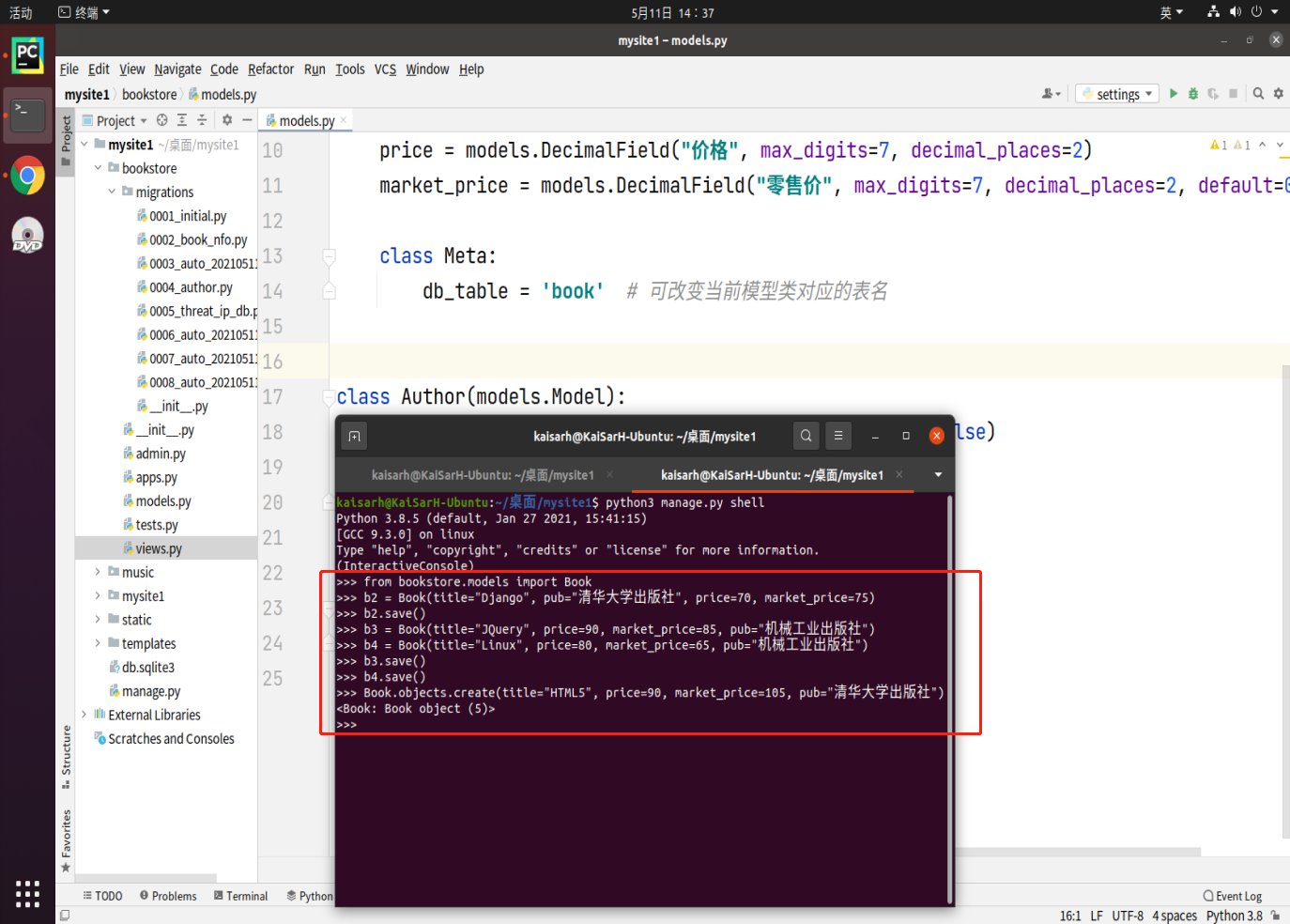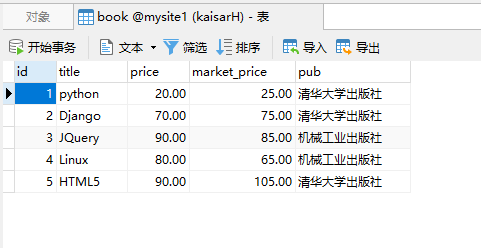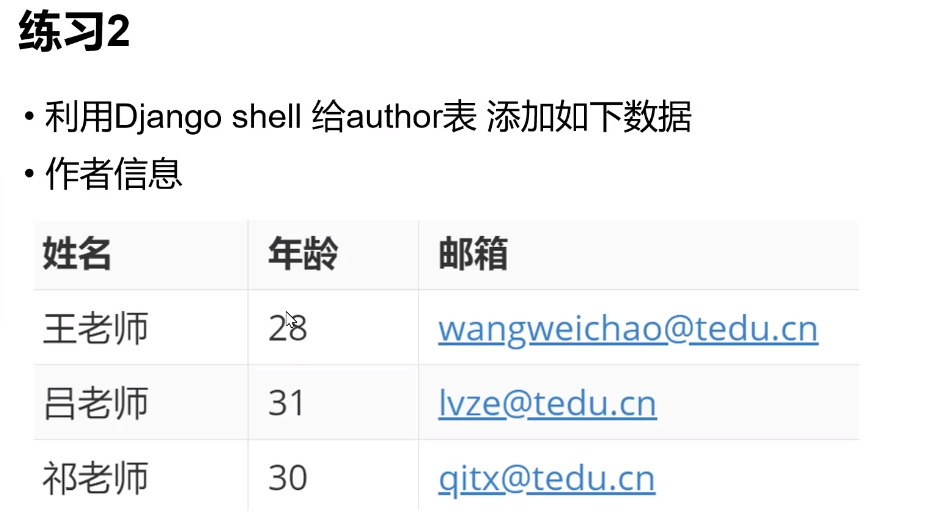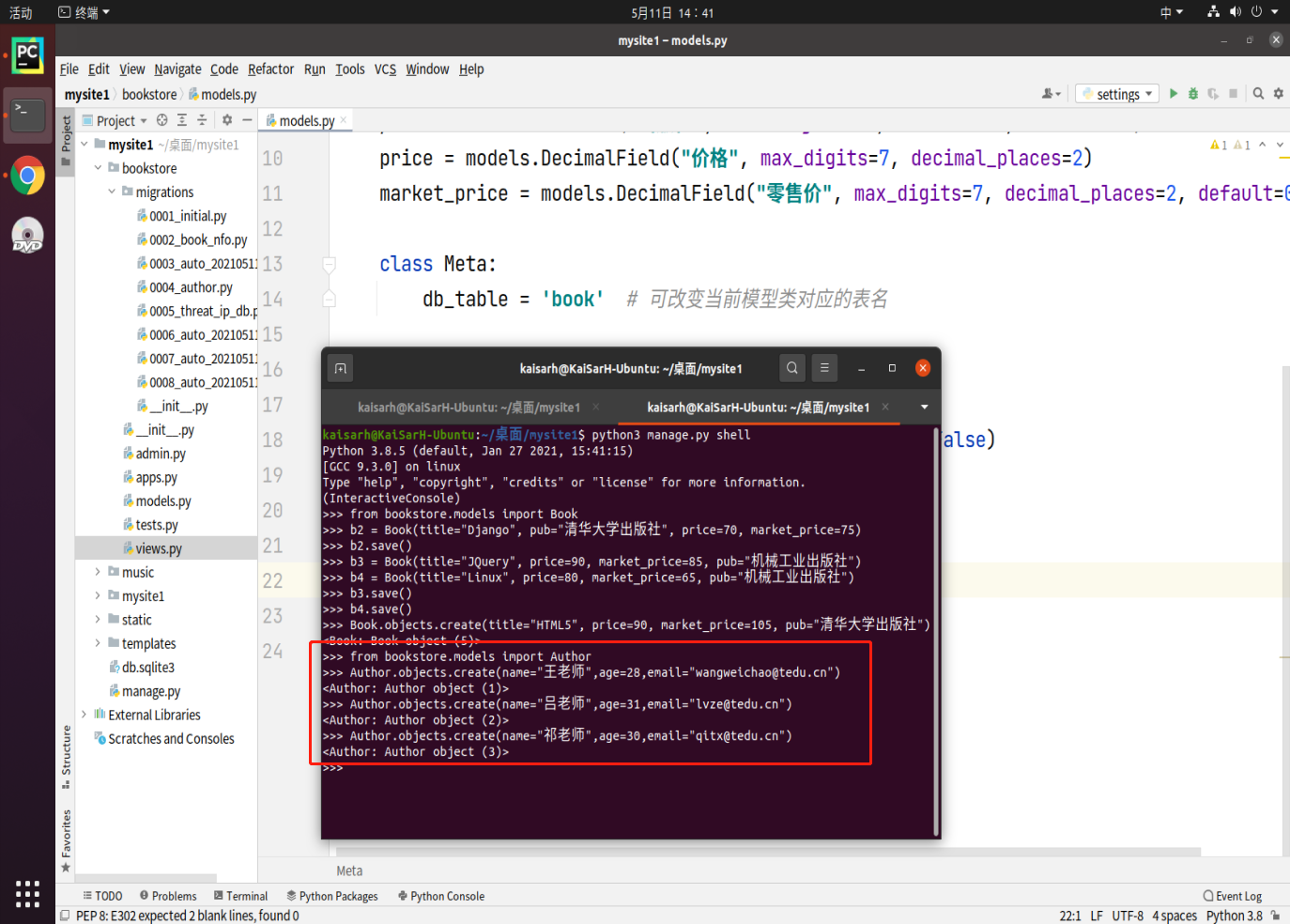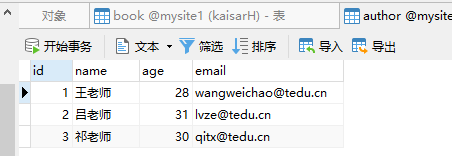## 6.3. 查询数据

### 6.3.1. 查询简介

• 数据库的查询需要使用管理器对象进行
• 通过MyModel.objects管理器方法调用查询方法

all()查询全部记录，返回QuerySet查询对象
get()查询符合条件的单一记录
filter()查询符合条件的多条记录
exclude()查询符合条件之外的全部记录
···

### 6.3.2. all()方法

``````from bookstore.models import Book

books = Book.objects.all()
for book in books:
print("书名", book.title, "出版社", book.pub)
``````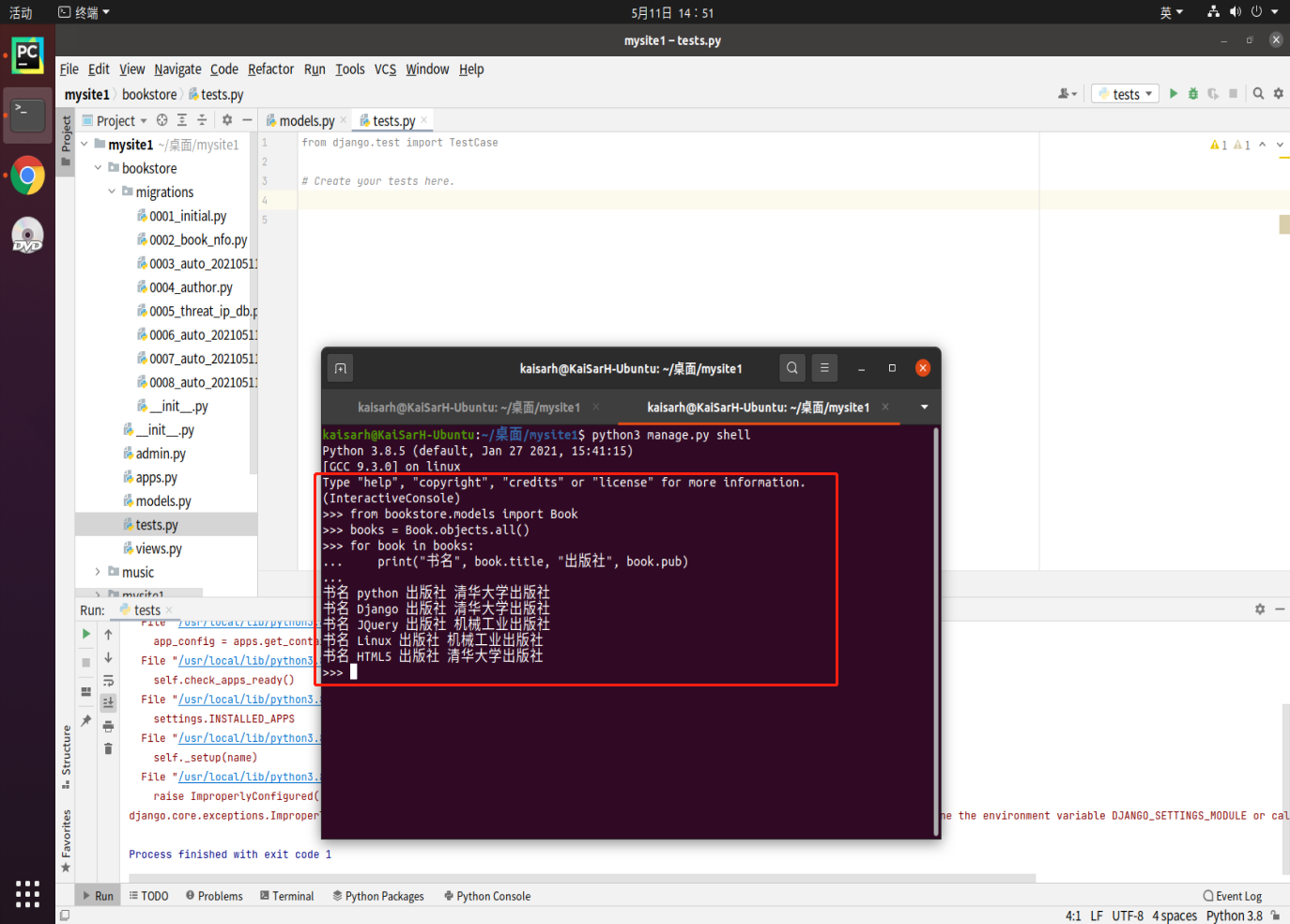### 6.3.3. 查询技巧

``````def __str__(self):
return "%s_%s_%s_%s"%(self.title,self.price,self.pub,self.market_price)
``````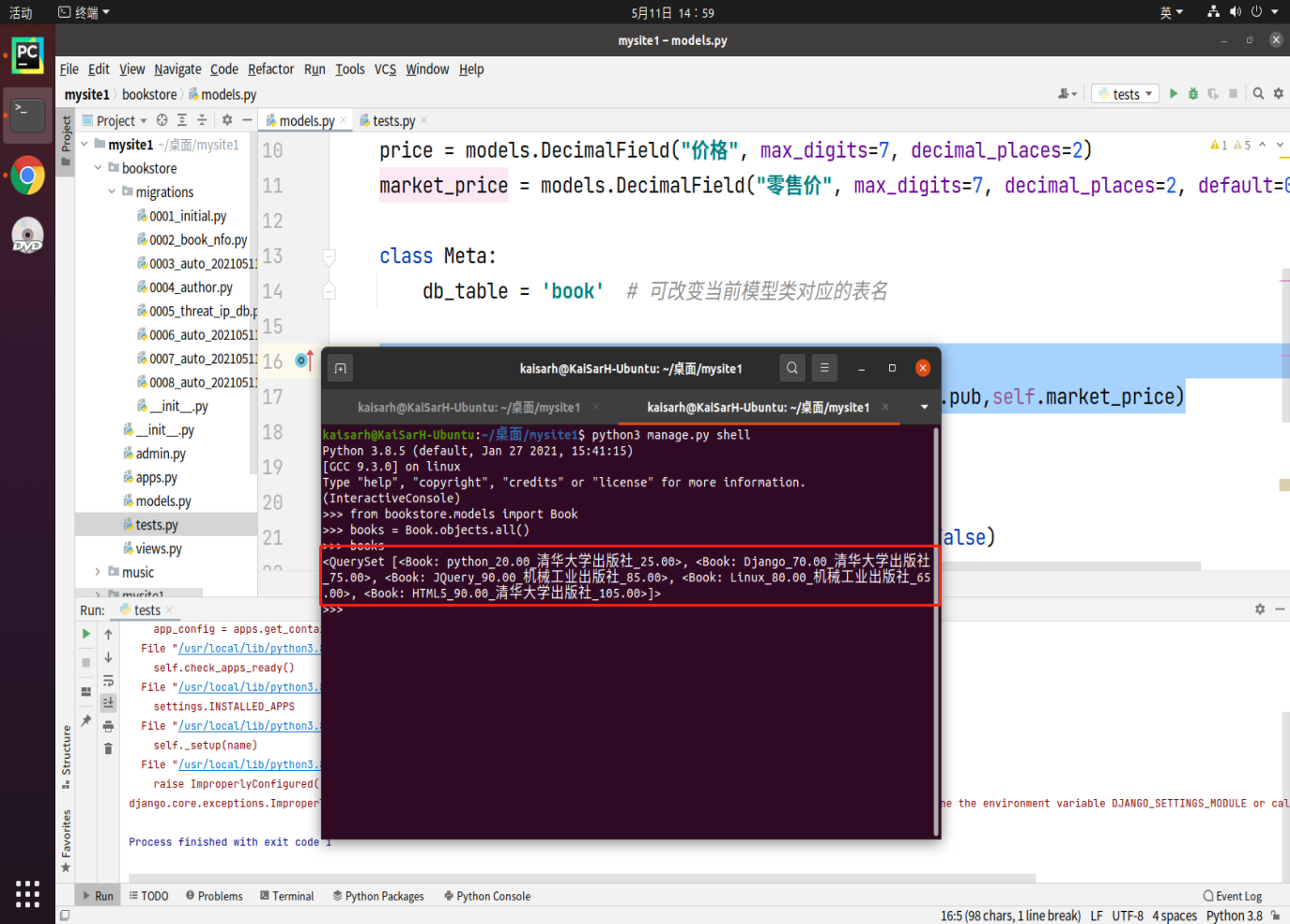### 6.3.4. values(…)

``````MyModel.objects.values('列1','列2'..)
``````

``````{
"列1":值1,
"列2":值2
}
``````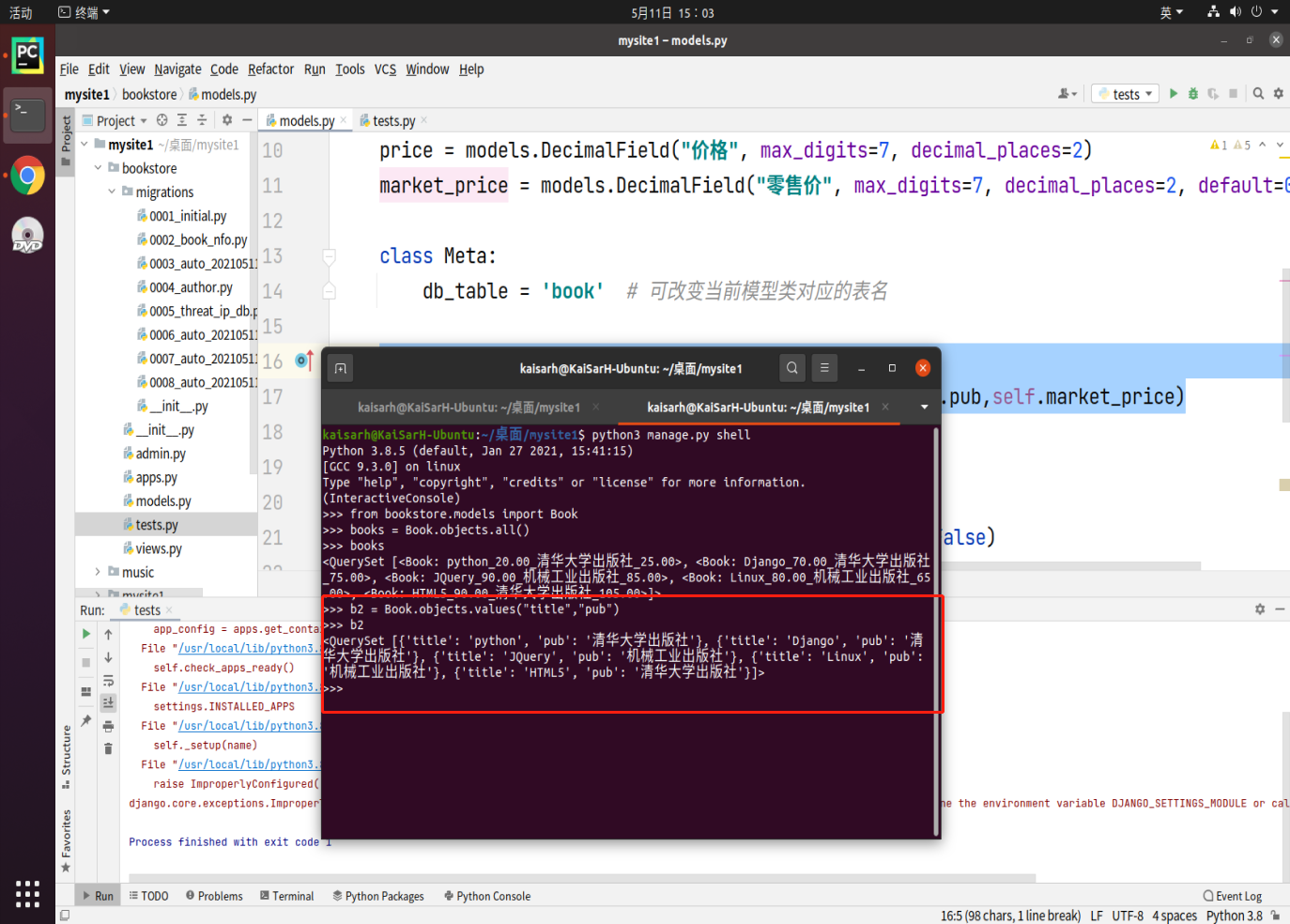### 6.3.5. values_list(…)

``````MyModel.objects.values_list('列1','列2'...)
``````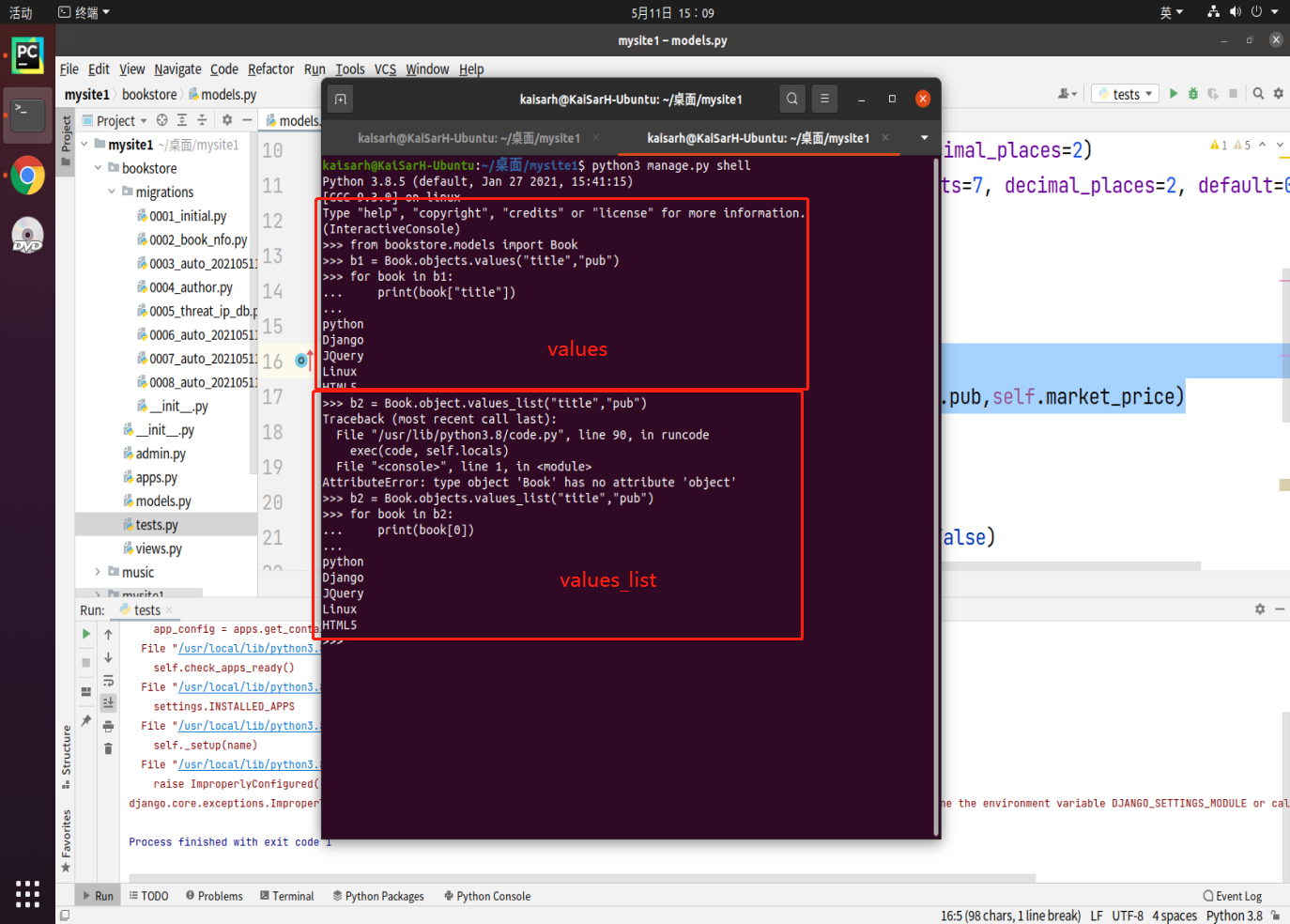### 6.3.6. order_by()

``````MyModel.objects.order_by('-列','列')
``````

• 默认是按照升序排序，降序排序则需要在列前面增加`'-'`表示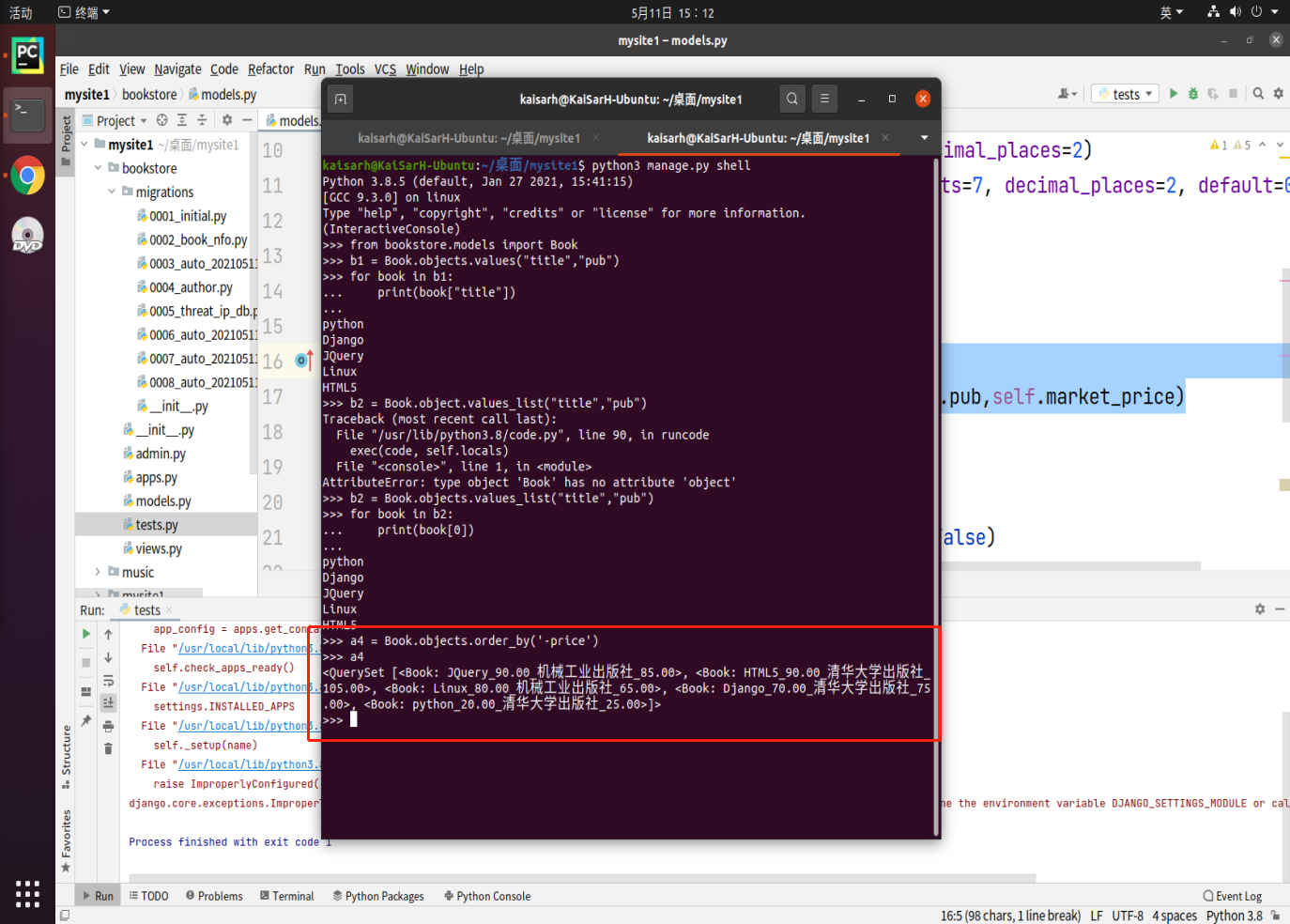• 方法之间支持链路调用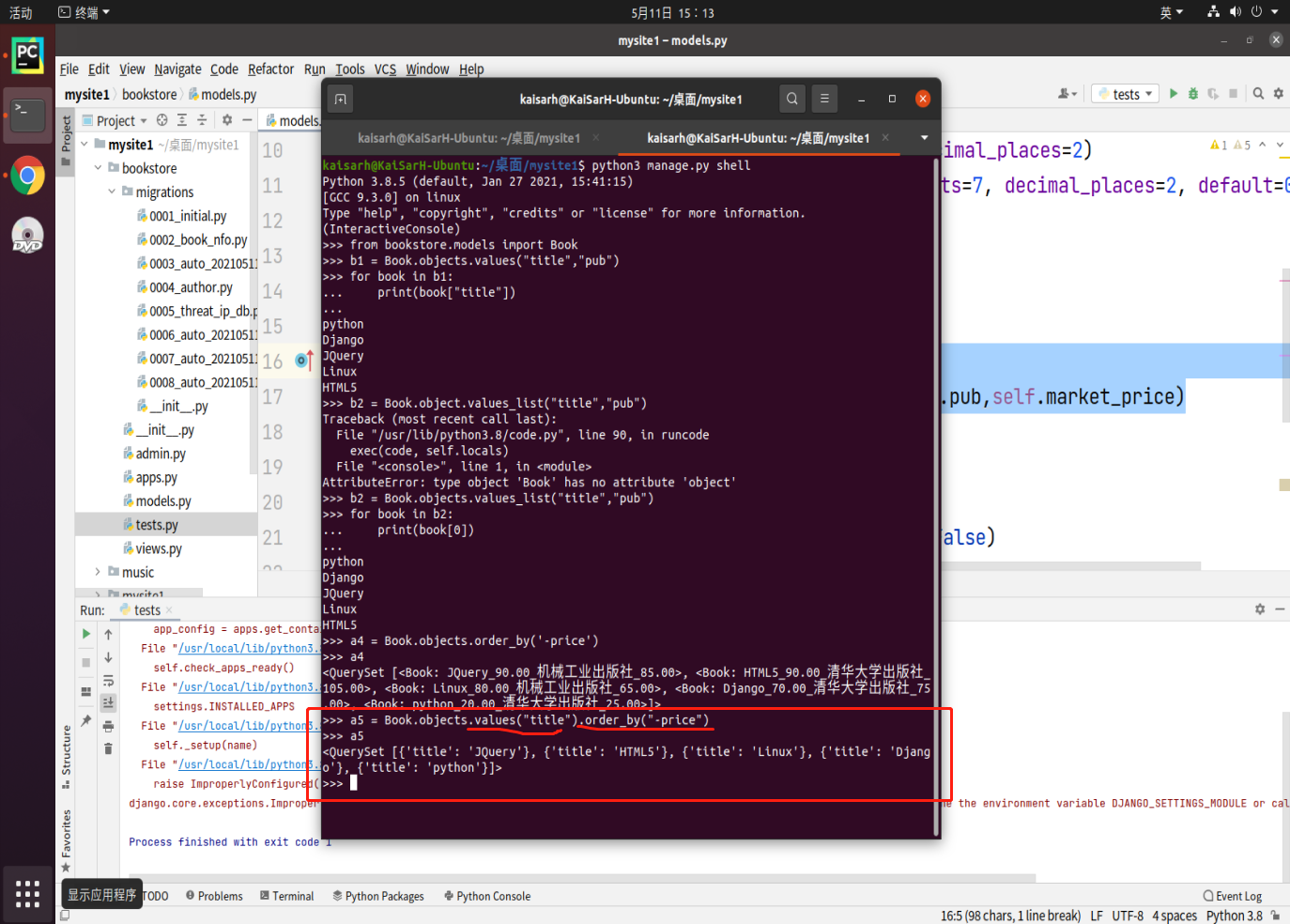### 6.3.7. query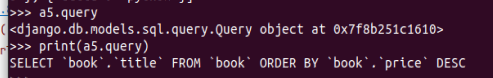### 6.3.8. 练习1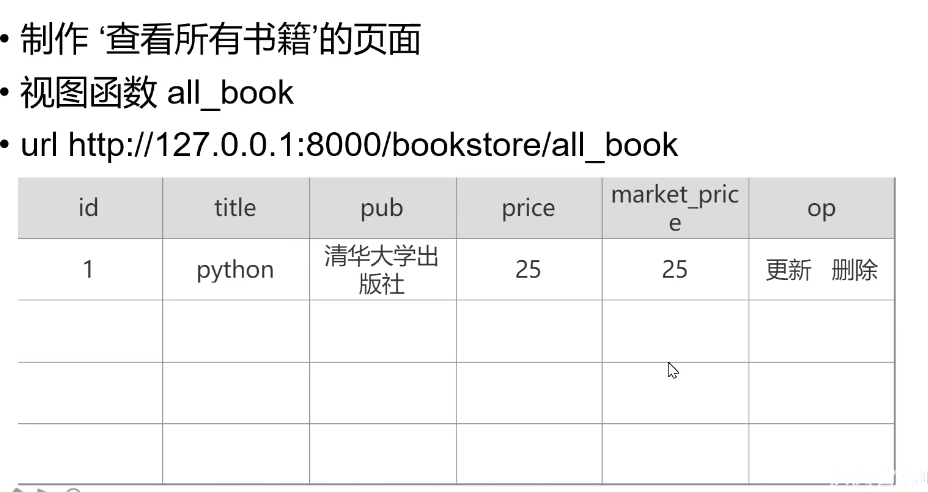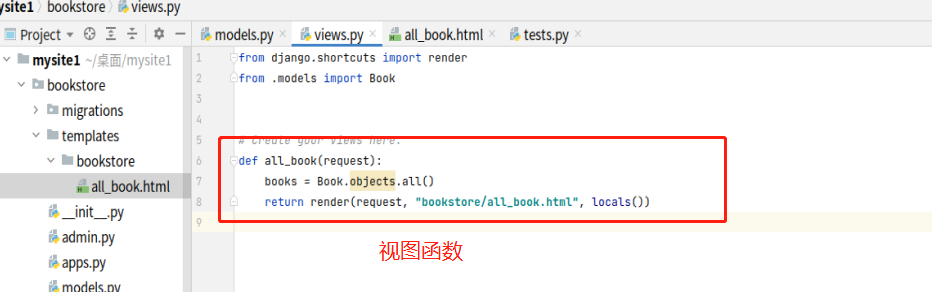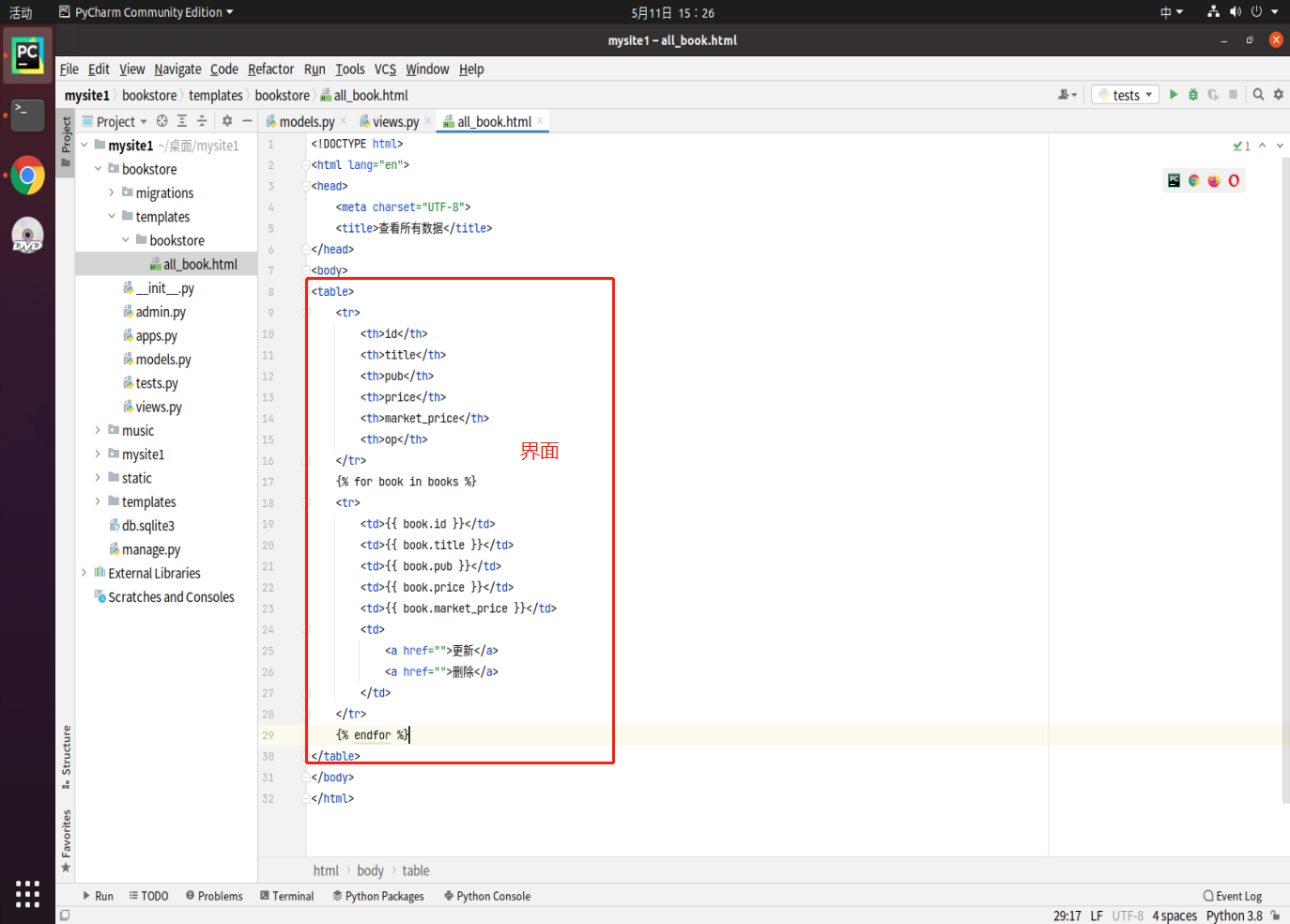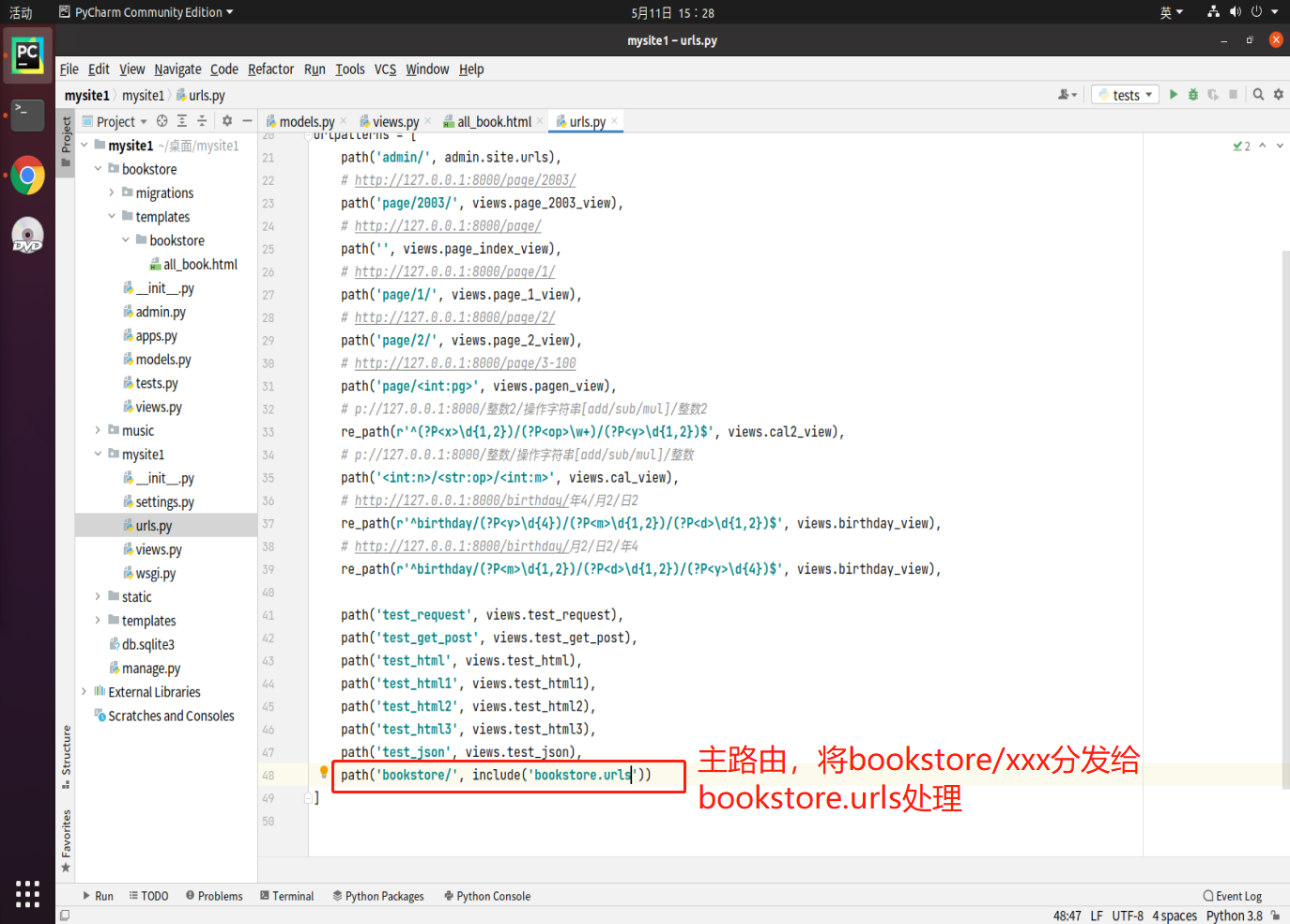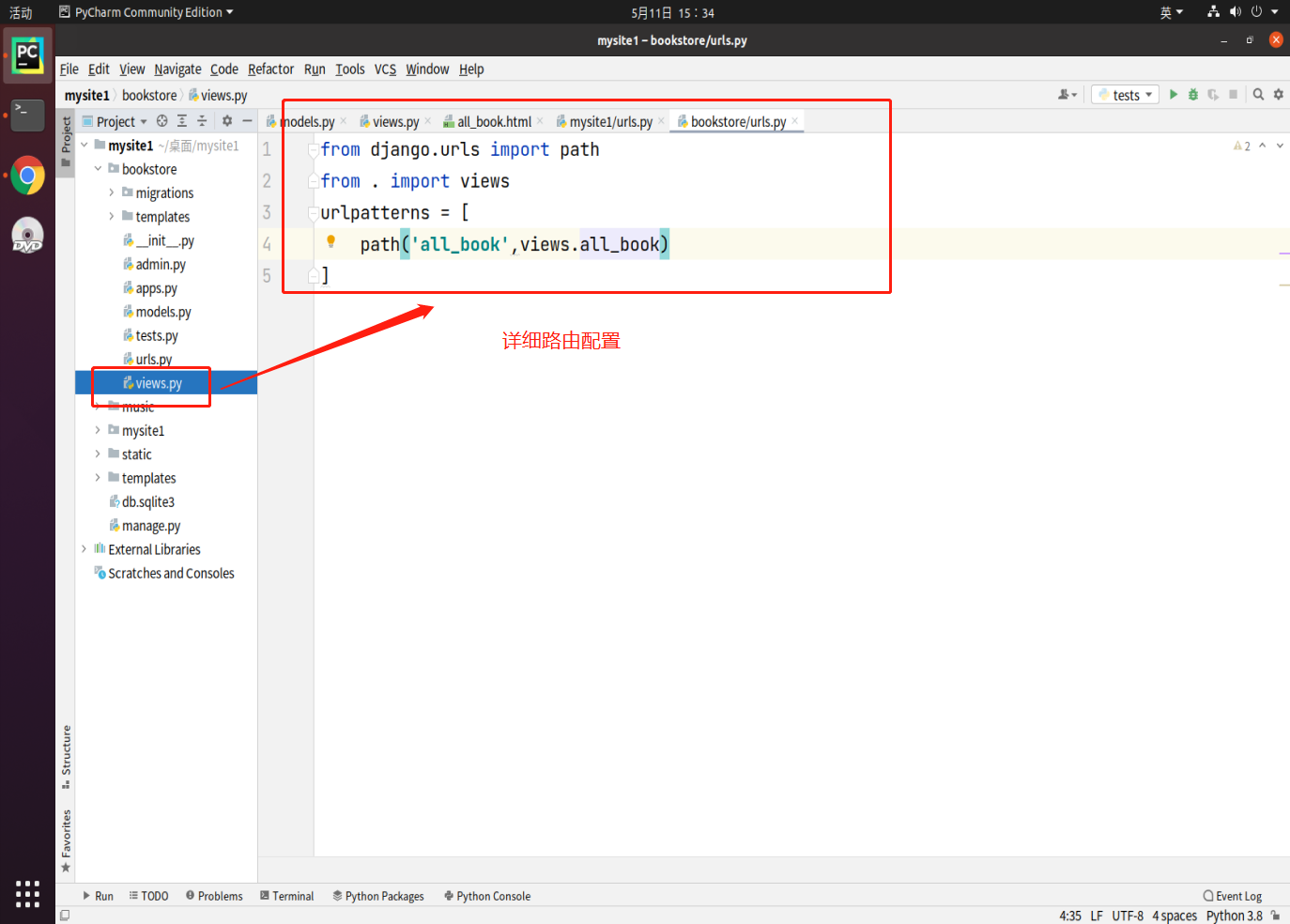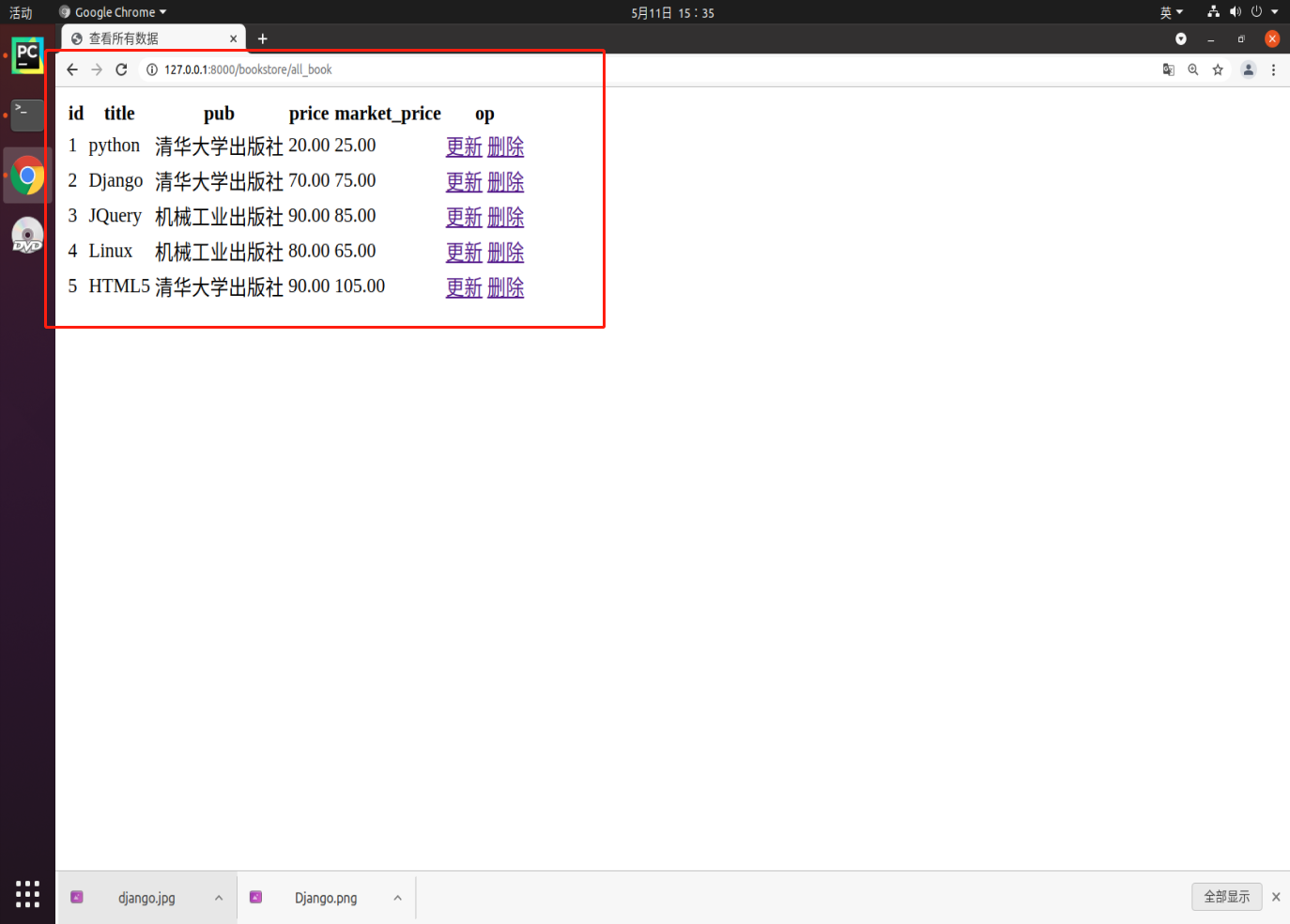### 6.3.9. filter(条件)

``````MyModel.objects.filter(属性1=值1,属性2=值2...)
``````

``````# 查询书中出版社为"清华大学出版社"的图书
from bookstore.models import Book
books = Book.objects.filter(pub = "清华大学出版社")
for book in books:
print("书名:", book.title)

# 查询Author实体中name为王老师并且age是28岁的
from bookstore.models import Author
author = Author.objects.filter(name='王老师', age=28)
``````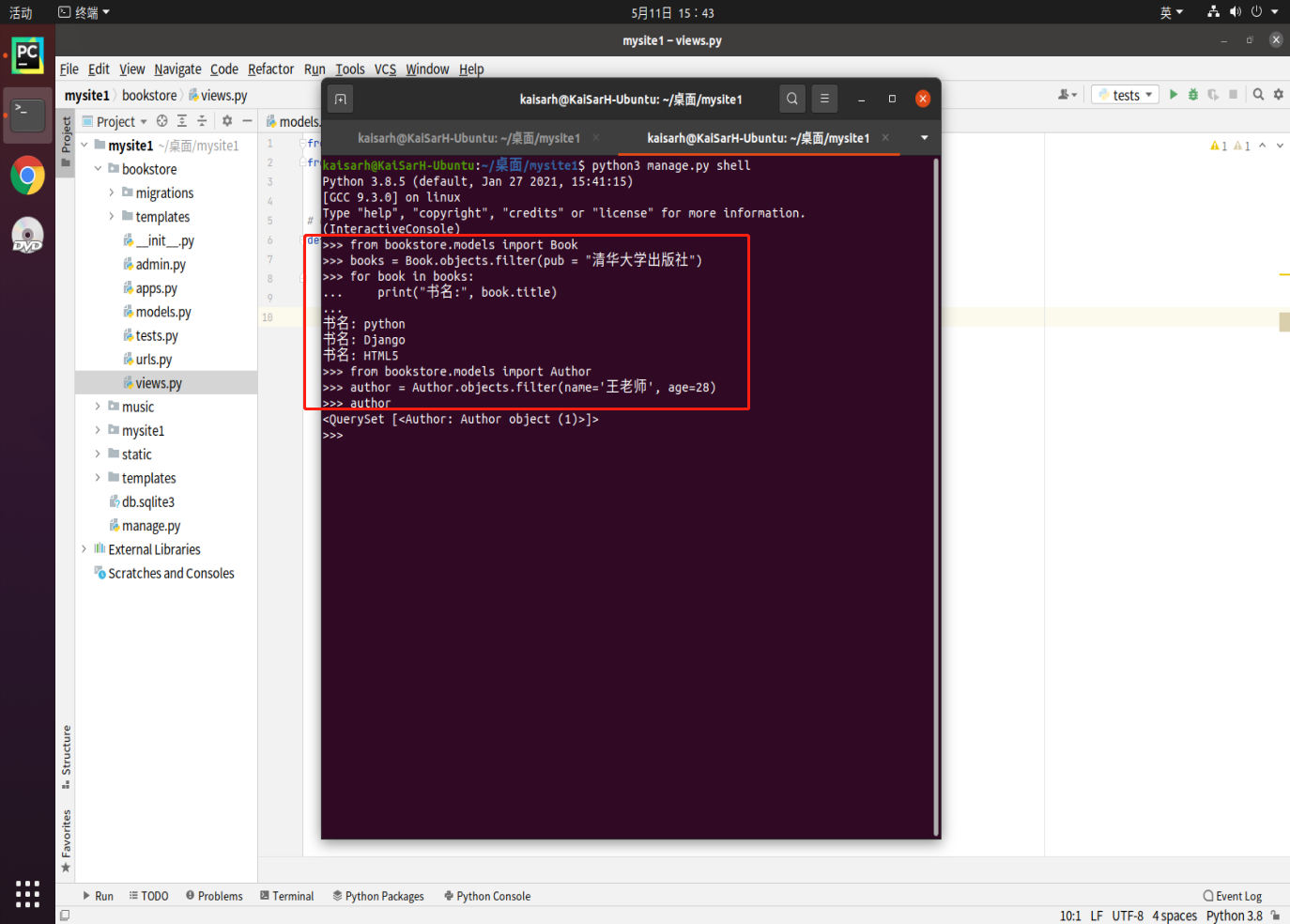### 6.3.10. exclude(条件)

``````MyModel.objects.exclude(条件)
``````

``````# 查询清华大学出版社,定价等于50以外的全部图书
from bookstore.models import Book
books = Book.objects.exclude(pub="清华大学出版社", price=50)
for book in books:
print(book)
``````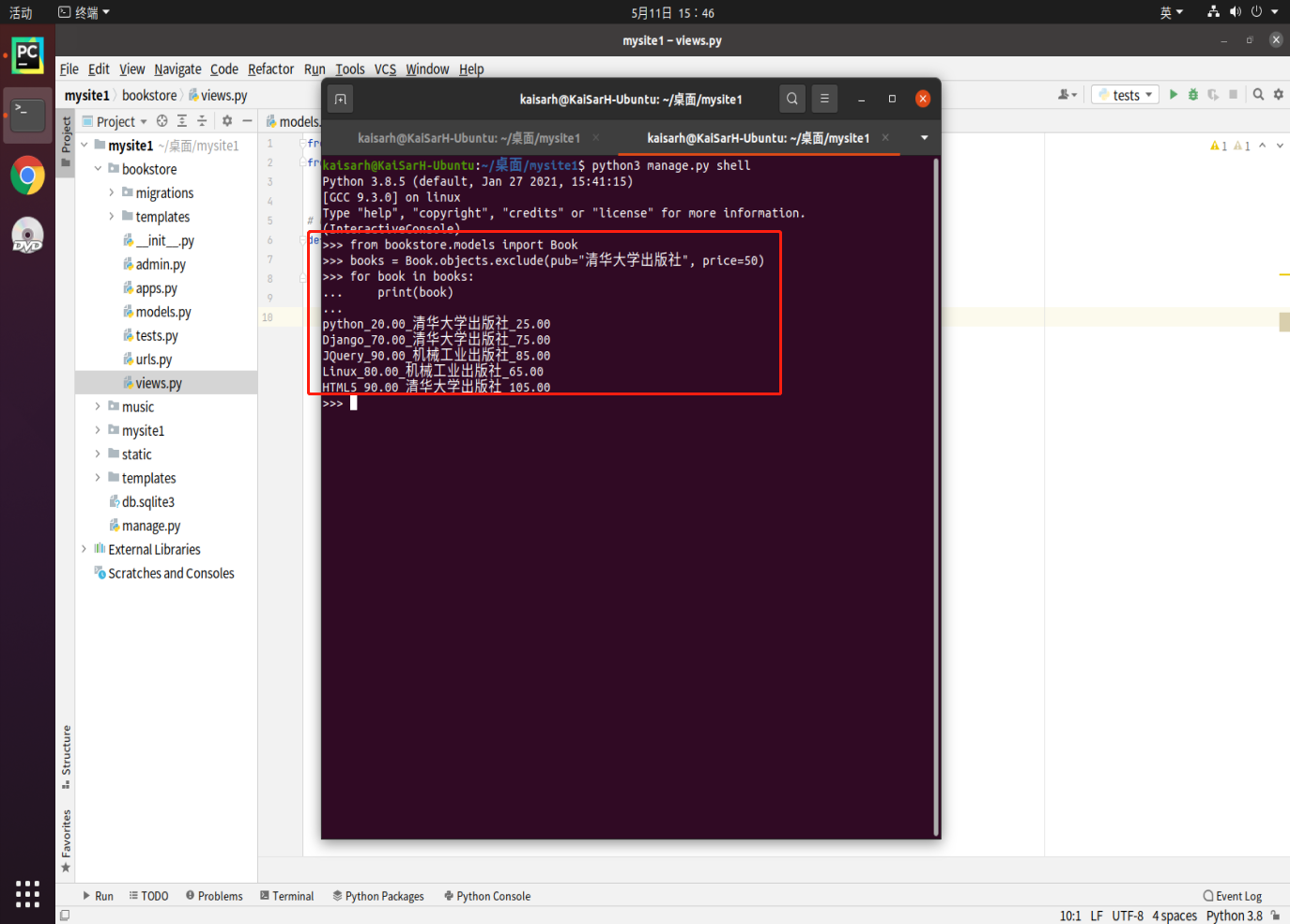### 6.3.11. get(条件)

``````MyModel.objects.get(条件)
``````

### 6.3.12. 查询谓词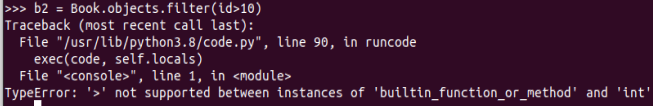__exact等值查询Author.objects.filter(id__exact=1)select * from author where id = 1
__contains包含指定值Author.objects.filter(name__contains=‘w’)select * from author where name like ‘%w%’
__startswith以XXX开始Author.objects.filter(name__startswith=‘w’)select * from author where name like ‘w%’
__endswith以XXX结束Author.objects.filter(name__endswith=‘w’)select * from author where name like ‘%w’
__gt大于指定值Author.objects.filter(age__gt=50)select * from author where age > 50
__gte大于等于指定值Author.objects.filter(age__gte=30)select * from author where age >= 50
__lt小于指定值Author.objects.filter(age__lt=30)select * from author where age < 50
__lte大于等于指定值Author.objects.filter(age__lte=30)select * from author where age <= 50
__in查询数据是否在指定范围内Author.objects.filter(country__in=[‘中国’,‘日本’,‘韩国’])select * from author where country in (‘中国’,‘日本’,‘韩国’)
__range查找数据是否在指定区间范围内Author.objects.filter(age__range=(30,50))select * from author where age between 35 and 50

## 6.4. 更新数据

### 6.4.1. 单个数据

1. 查：通过`get()`得到要修改的实体对象
2. 改：通过`对象.属性`的方式修改数据
3. 存：通过`对象.save()`保存数据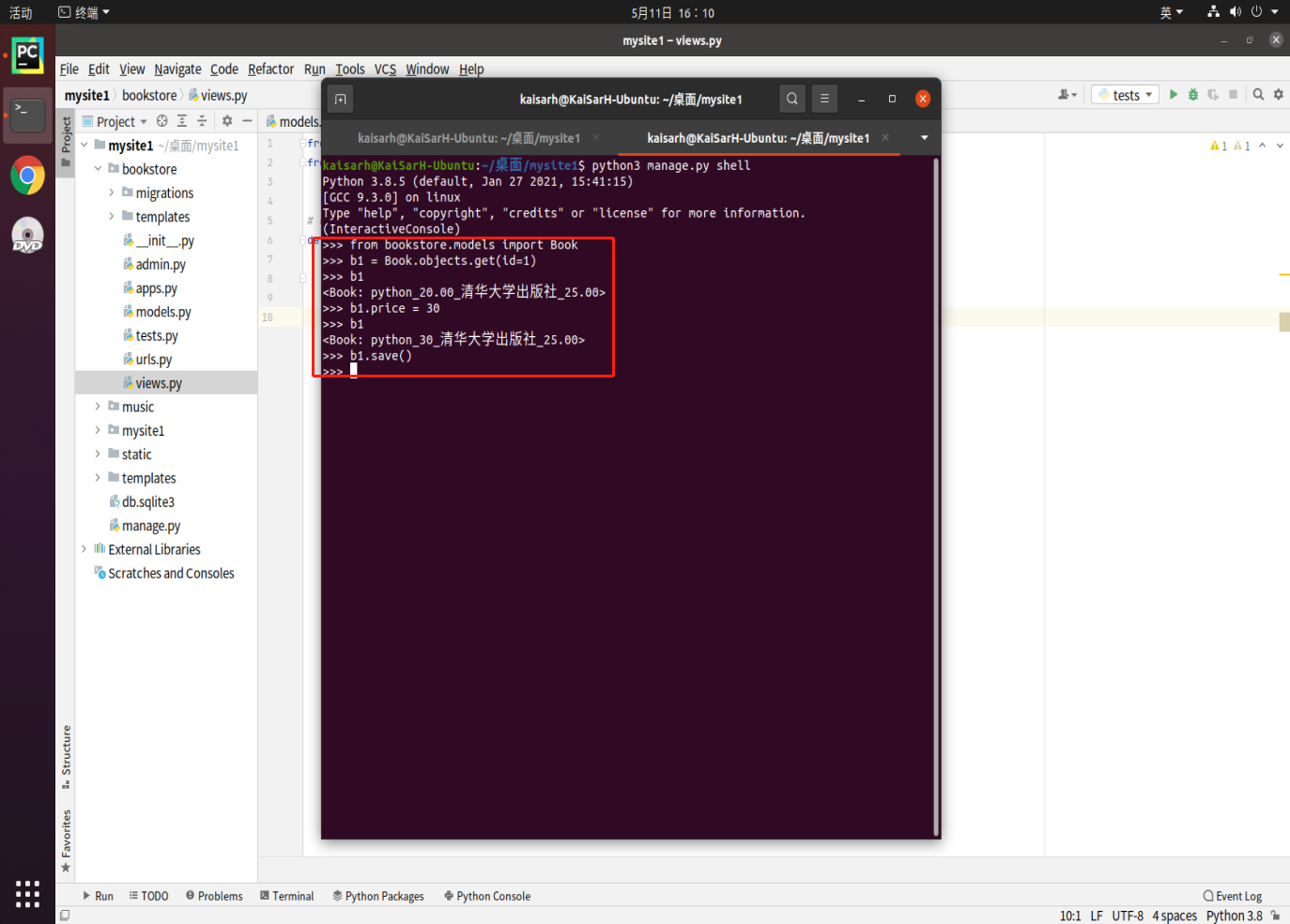### 6.4.2. 批量更新

``````# 将id大于3的所有图书价格定为0元
books = Book.objects.filter(id__gt=3)
books.update(price=0)
# 将所有书的零售价定为100元
books = Book.objects.all()
books.update(market_price=100)
``````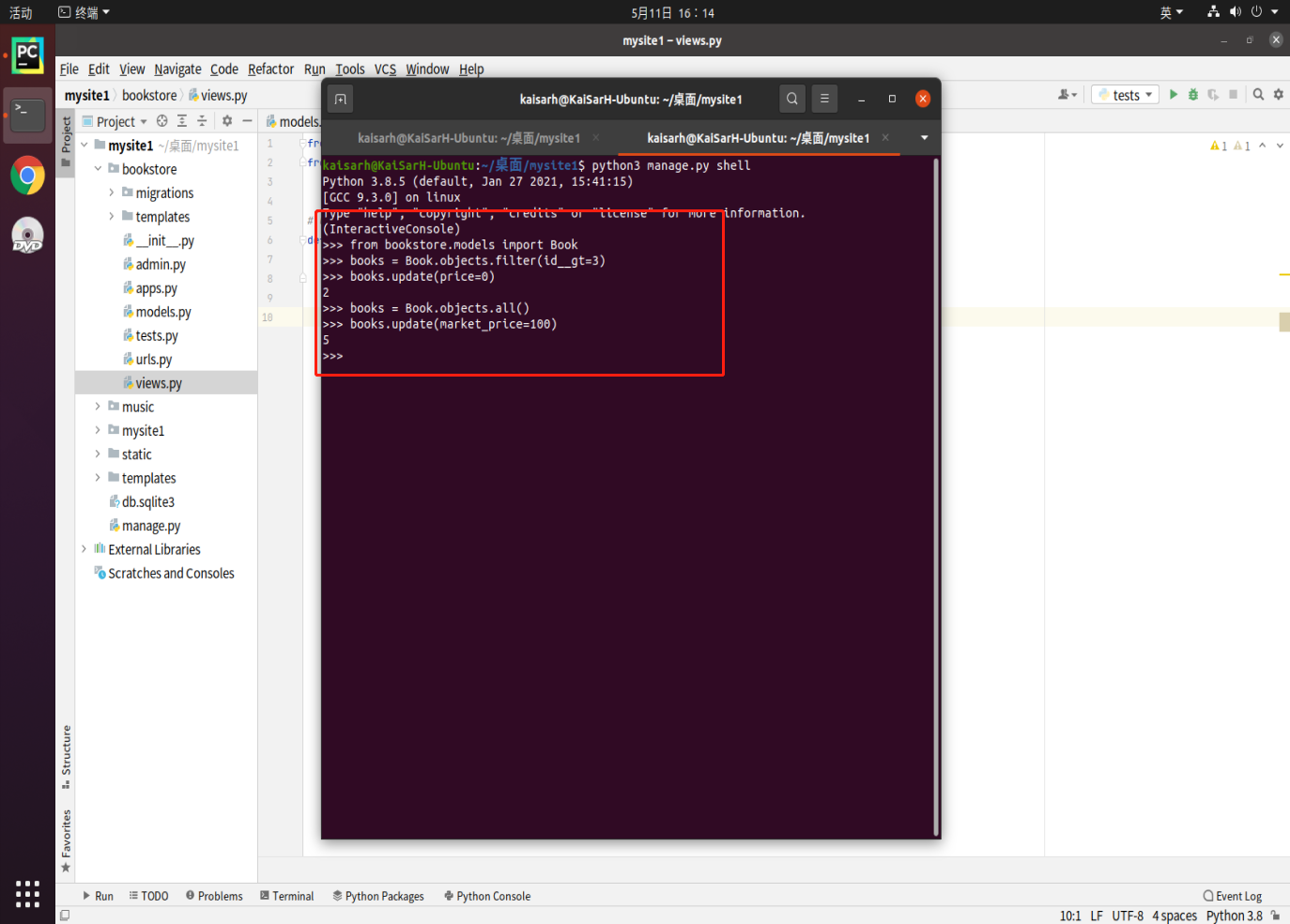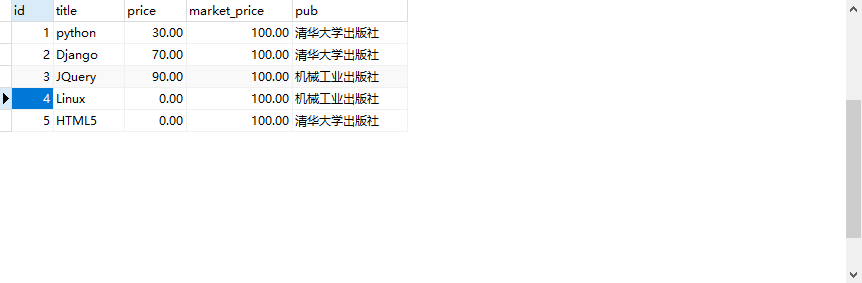### 6.4.3. 练习2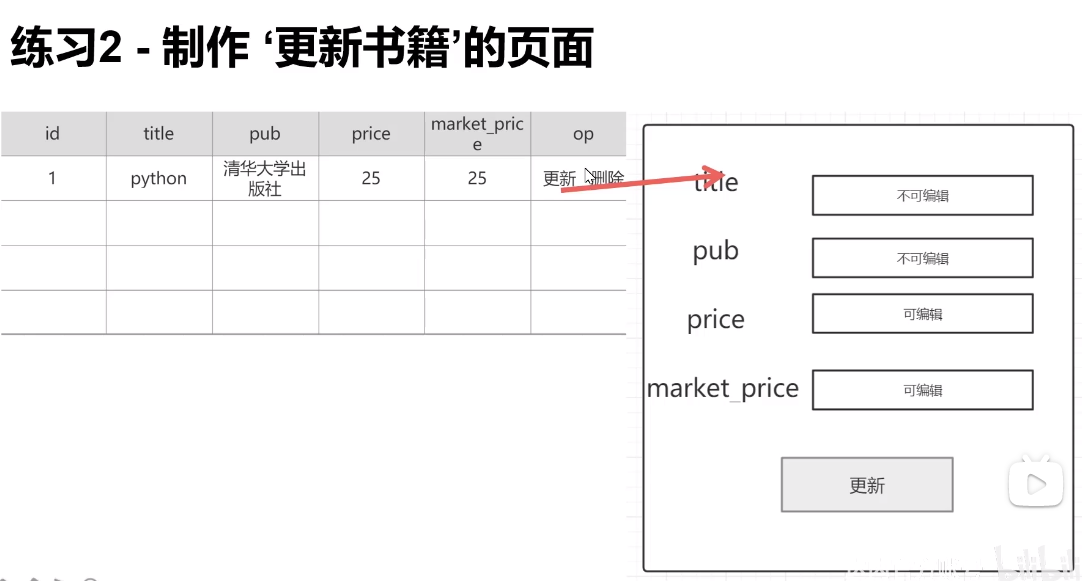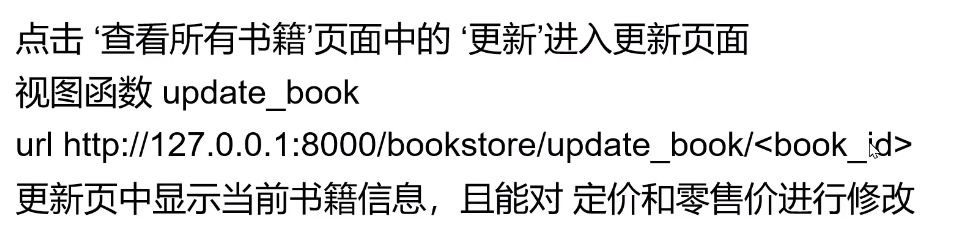• 列表界面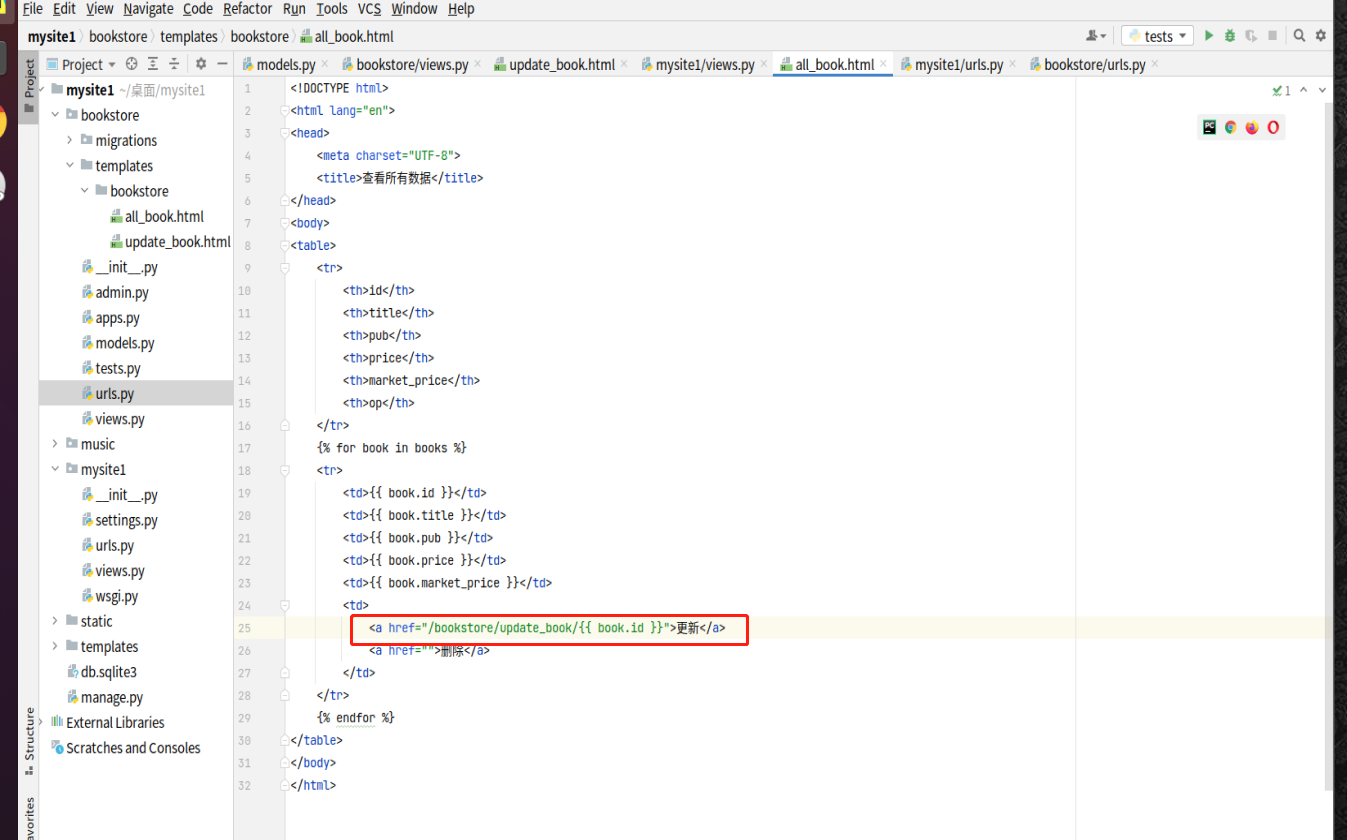• 更新界面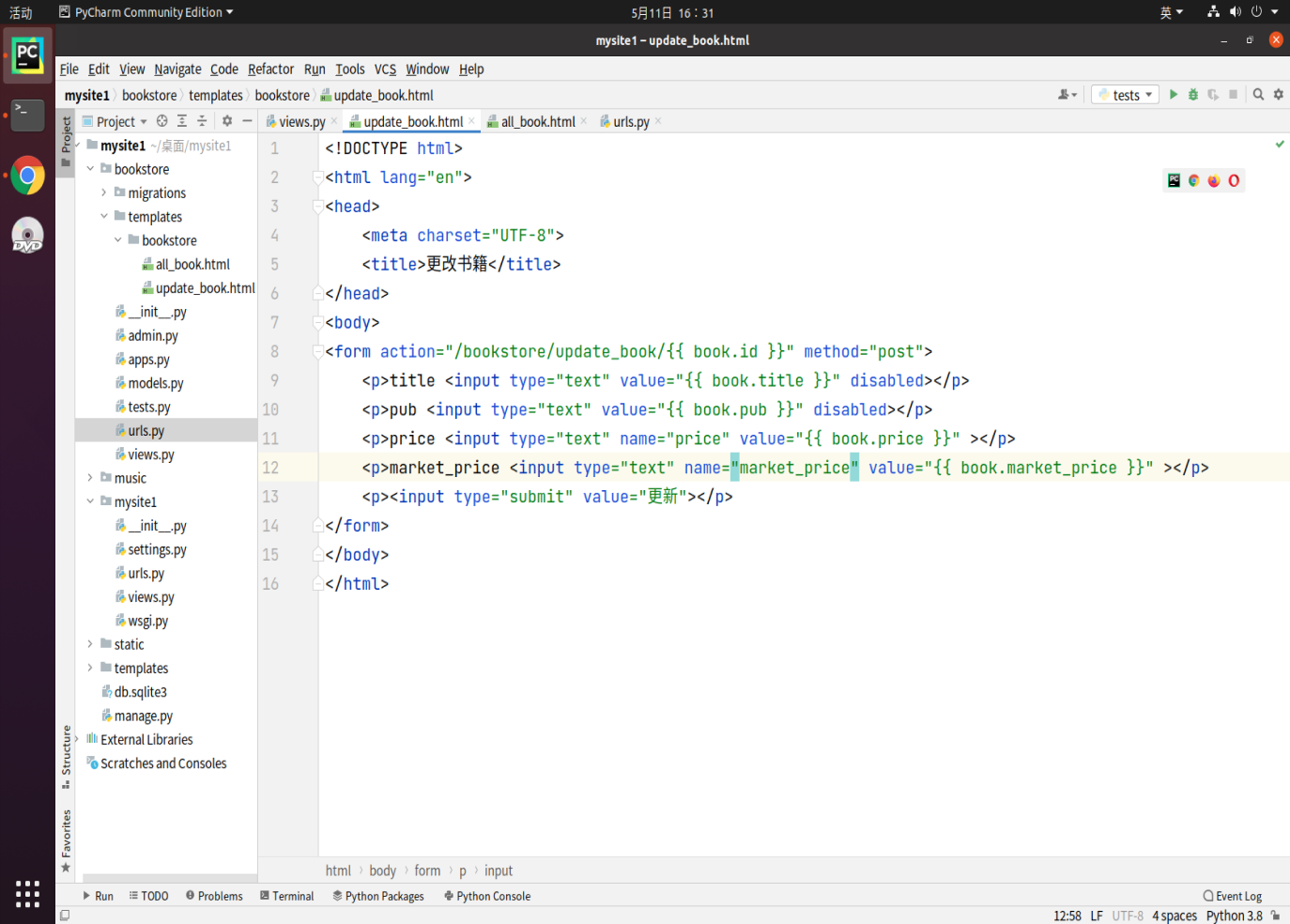• 视图函数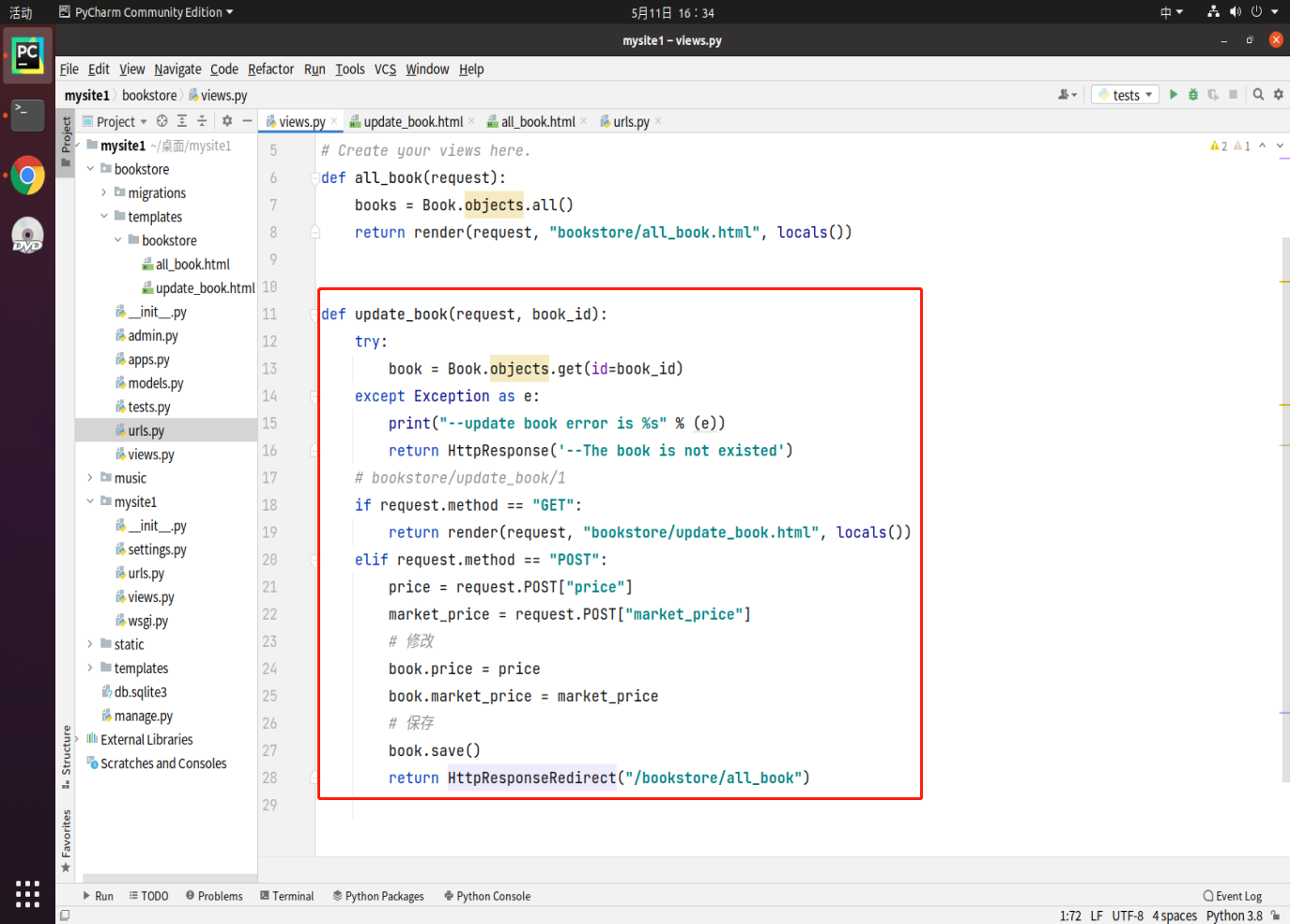• 路由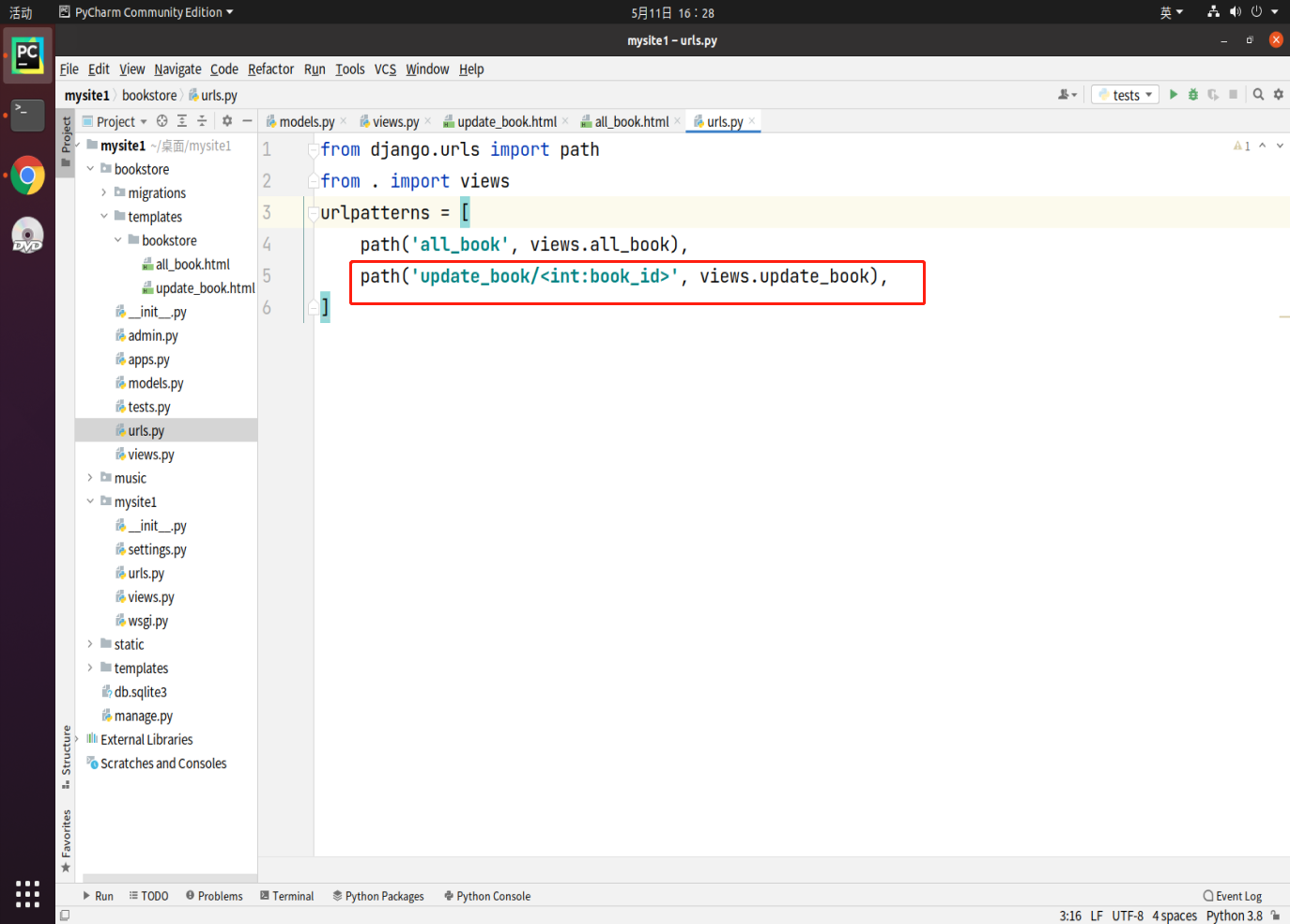## 6.5. 删除数据

### 6.5.1. 干个数据

1. 查找查询结果对应的一个数据对象
2. 调用这个数据对象的`delete()`方法实现删除
``````try:
auth = Author.objects.get(id=1)
auth.delete()
except:
print(删除失败)
``````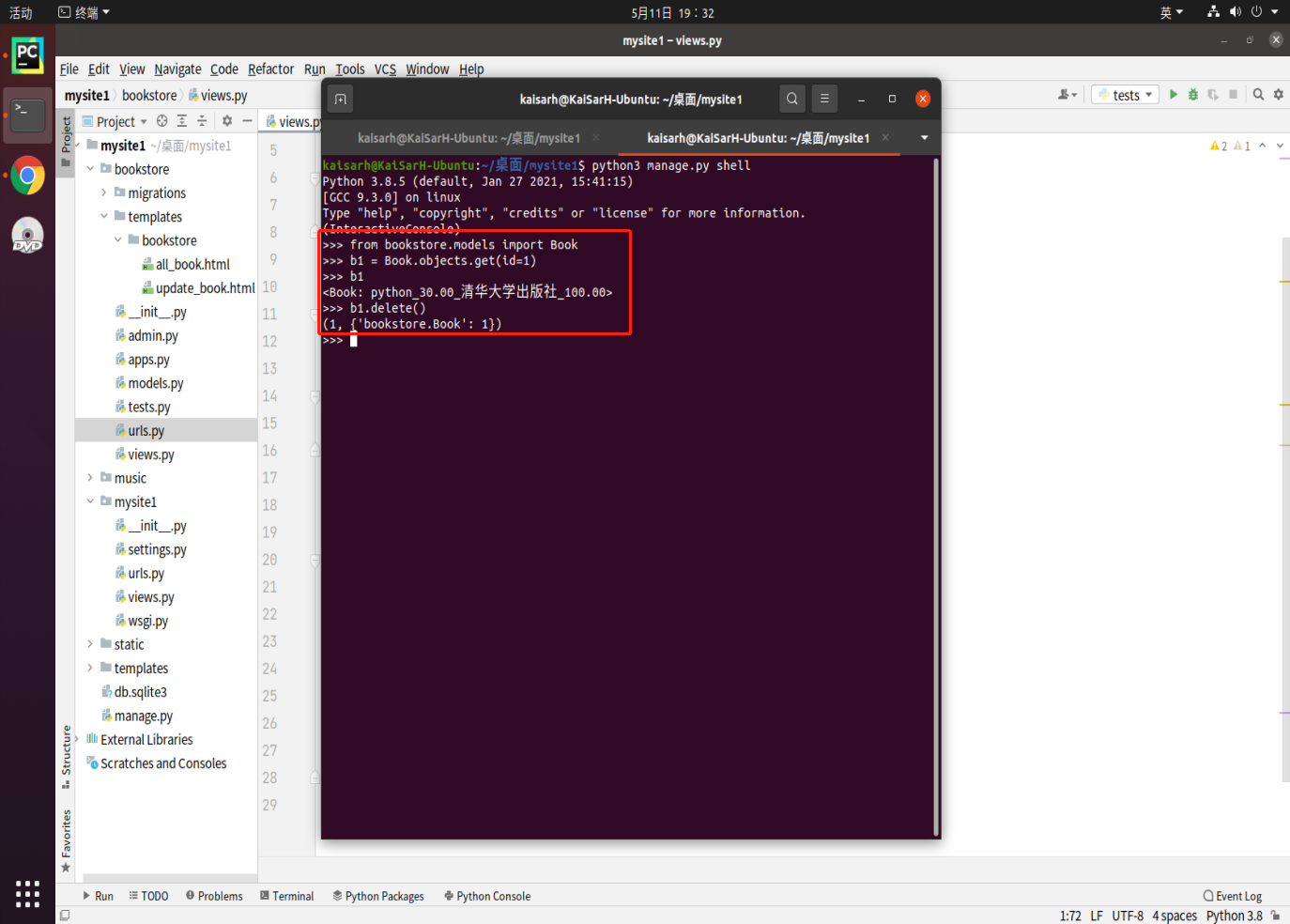### 6.5.2. 批量数据

1. 查找查询结果集中满足条件的全部QuerySet查询集合对象
2. 调用查询集合对象的delete()方法实现删除
``````# 删除全部作者中，年龄大于65的全部信息
auths = Author.objects.filter(age__gt=65)
auths.delete()
``````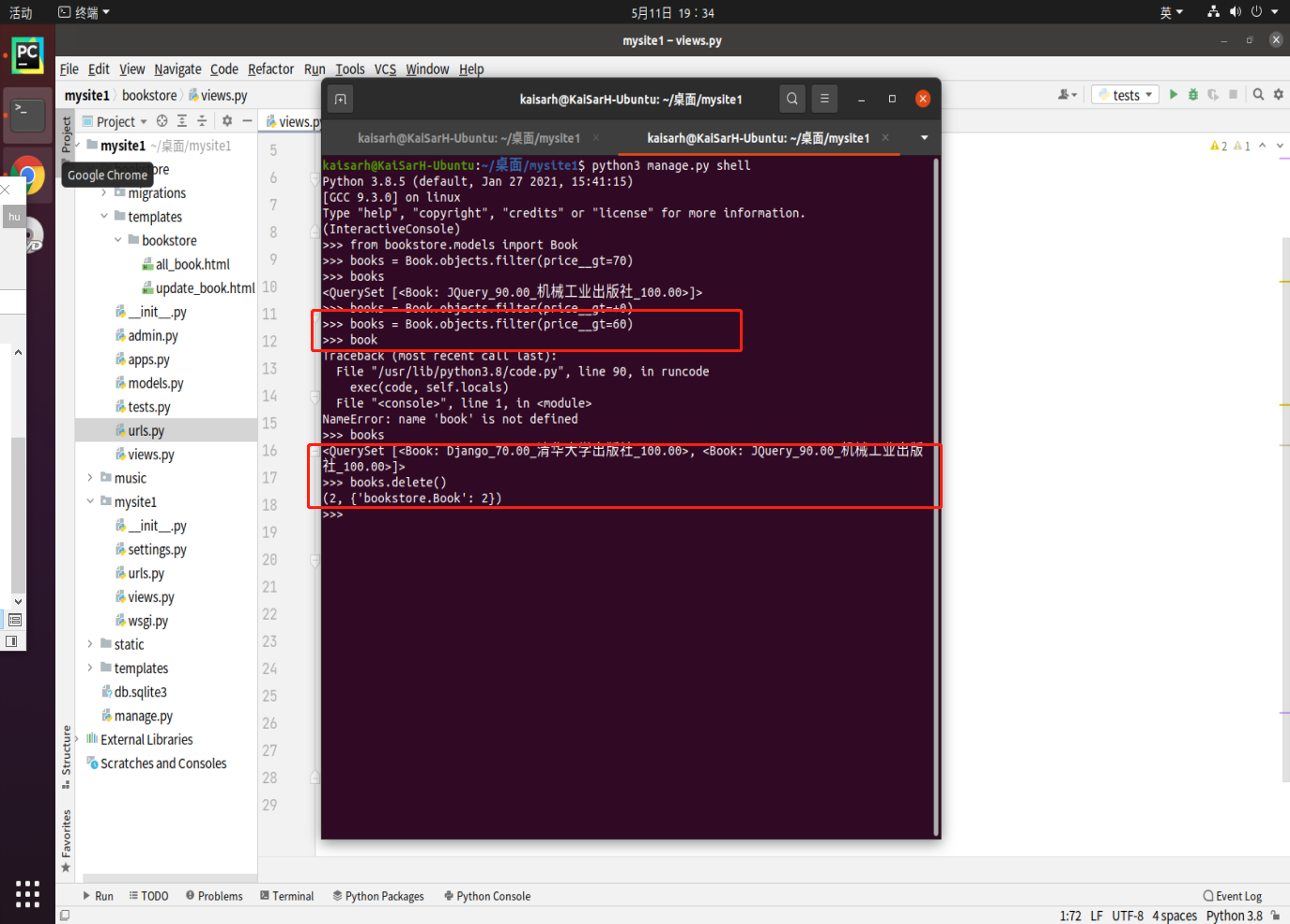### 6.5.3. 伪删除

• 通常不会轻易在业务中把数据真正删掉，取而代之的是做伪删除。

即在表中添加一个布尔型字段（is_active），默认是True；执行删除时，将欲删除数据的is_active字段置为False

• 注意：用伪删除时，确保显示数据的地方，均加了is_active = True的过滤查询

## 6.6. F对象和Q对象

### 6.6.1. F对象

• 通常是对数据库的字段值在不获取的情况下进行操作
• 用于类属性（字段）之间的比较

``````from django.db.models import F
F('列名')
``````

``````Book.objects.all().update(market_price=F('market_price')+10)
'UPDATE `bookstore_book` SET `market_price` = (`bookstore_book`.`market_price` + 10)'
# 以上代码好于如下代码
books = Book.objects.all()
for book in books:
book.market_price = book.market_price + 10
book.save()
``````

``````from django.db.models import F
from bookstore.models import Book
books = Books.objects.filter(market_price__gt=F('price'))
'SELECT * FROM `bookstore_book` WHERE `bookstore_book`.`market_prict` > `bookstore_book`.`price`'
for book in books:
print(book.title, "定价:", book.price, "现价:", book.market_price)
``````

### 6.6.2. Q对象

``````Book.objects.filter(Q(price__lt=20)|Q(pub="清华大学出版社"))
``````

Q对象在数据包 django.db.models中，需要先导入再使用。

& 与运算符

| 或运算符

``````from django.db.models import Q
Q(条件1)|Q(条件2) # 条件1成立或条件2成立
Q(条件1)&Q(条件2) # 条件1和条件2同时成立
Q(条件1)&~Q(条件2) # 条件1成立且条件2不成立
···
``````

## 6.7. 聚合查询

• 整表聚合
• 分组聚合

### 6.7.2. 整表聚合

• 导入方法：

``````from django.db.models import *
``````
• 聚合函数：

Sum、Avg、Count、Max、Min

• 语法：

``````MyModel.objects.aggregate(结果变量名=聚合函数('列'))
``````
• 返回结果：结果变量名和值组成的字典

格式为：

``````{
"结果变量名":值
}
``````
• 示例：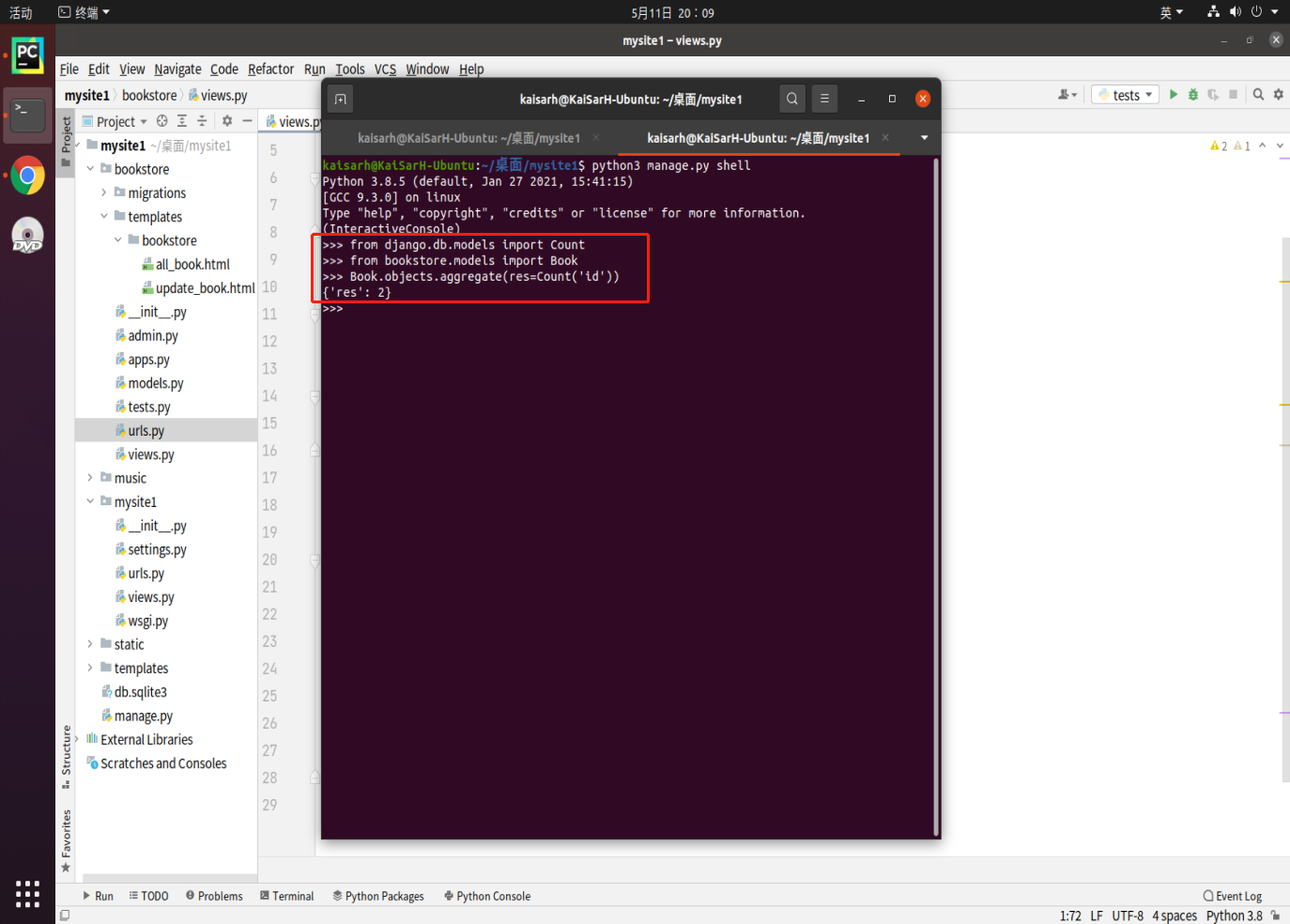### 6.7.3. 分组聚合

• 语法：

``````QuerySet.annotate(结果变量名=聚合函数('列'))
``````
• 返回值：QuerySet

• 流程：

1. 通过先用查询结果MyModel.objects.values查找查询要分组聚合的列

MyModel.objects.values(‘列1’,‘列2’)

如：

``````pub_set = Book.objects.values('pub')
print(pub_set) # <QuerySet [{'pub':'清华大学出版社'},{'pub':'清华大学出版社'},{'pub_hou'{'pub':'机械工业出版社'},{'pub'}:'清华大学出版社'}]>
``````
2. 通过返回结果的QuerySet.annotate方法分组聚合得到分组结果

QuerySet.annotate(名=聚合函数(‘列’))

``````pub_count_set = pub_set.annotate(myCount=Count('pub'))
print(pub_count_set) # <QuerySet [{'pub':'清华大学出版社','myCount':7},{'pub':'机械工业出版社', 'myCount':3}]
``````

# 七、原生数据库操作

Django也可以支持直接使用sql语句的方式通信数据库

``````MyModel.objects.raw(sql语句,拼接参数)
``````

RawQuerySet集合对象【只支持基础操作，比如循环】

``````books = Book.objects.raw('select * from book')
for book in books:
print(book)
``````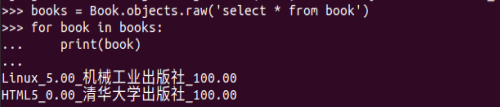``````s1 = Book.objects.raw('select * from book where id = %s'%('1 or 1=1'))
``````

sql注入防范：

``````s1 = Book.objects.raw('select * from book where id = %s'%('4 or 1=1'))
for s in s1:
print(s)
``````

``````s1 = Book.objects.raw('select * from book where id = %s',['4 or 1=1'])
for s in s1:
print(s)
``````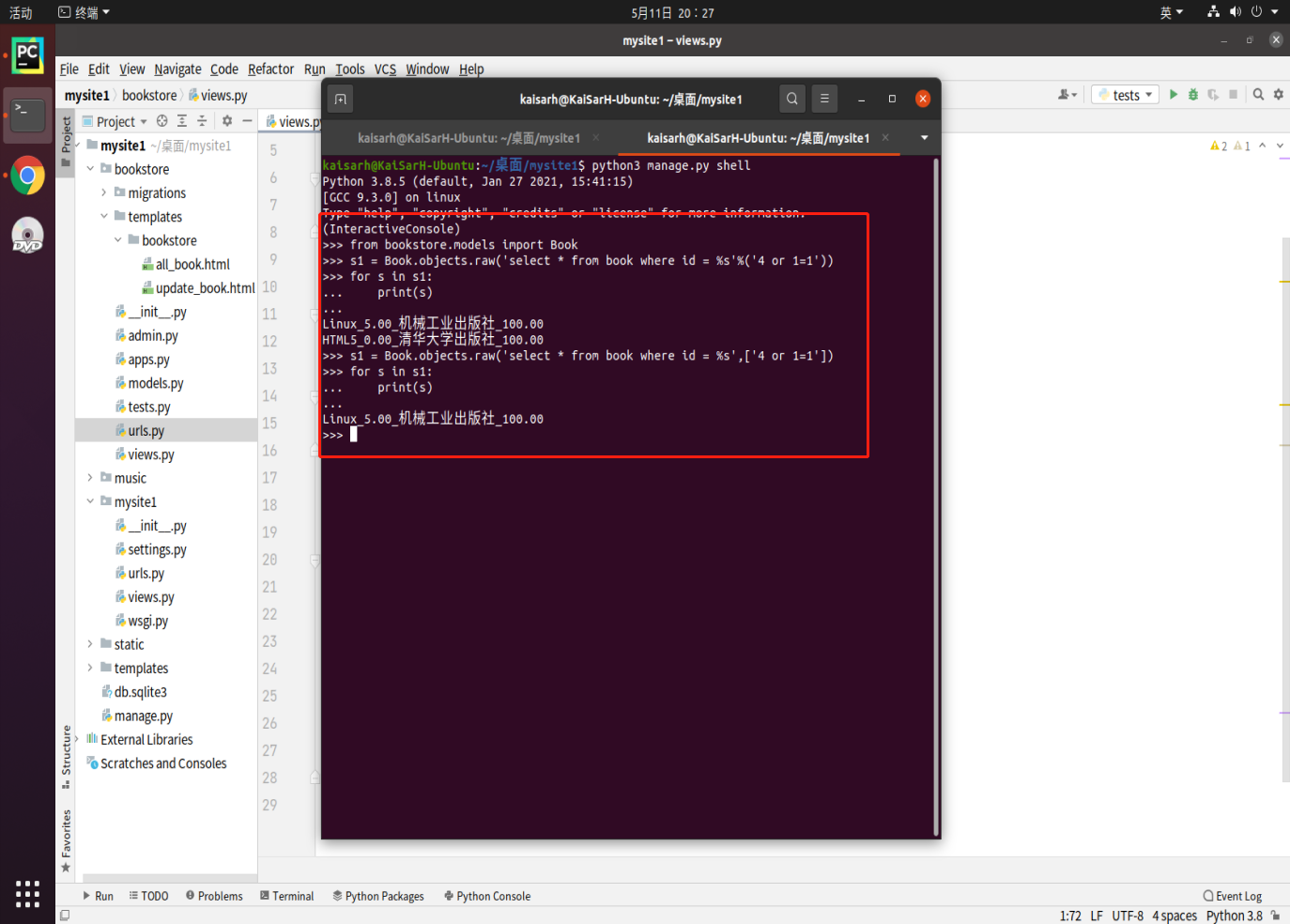# 八、cursor

1. 导入cursor所在的包

``````from django.db import connection
``````
2. 用创建cursor类的构造函数创建cursor对象，再使用cursor对象，为保证在出现异常时能释放cursor资源，通常使用with语句进行创建操作

``````from django.db import connection
with connection.cursor() as cur:
cur.execute('执行SQL语句','拼接参数')
``````
3. 示例：

``````# 用SQL语句将id为4的书的出版社改为"xxx出版社"
from django.db import connection
with connection.cursor() as cur:
cur.execute('update book set pub_house="xxx出版社" where id = 4;')
# 删除id为1的一条记录
with connection.cursor() as cur:
cur.execute('delete from book where id = 1;')
``````

25 0

pdf(new) 更多>